# Spatial analysis of NYC Shootings using the SPPT

As a follow up to my prior post on spatial sample size recommendations for the SPPT test, I figured I would show an actual analysis of spatial changes in crime. I’ve previously written about how NYC shootings appear to be going up by a similar amount in each precinct. We can do a similar analysis, but at smaller geographic spatial units, to see if that holds true for everywhere.

The data and R code to follow along can be downloaded here. But I will copy-paste below to walk you through.

So first I load in the libraries I will be using and set my working directory:

``````###################################################
library(sppt)
library(sp)
library(raster)
library(rgdal)
library(rgeos)

my_dir <- 'C:\\Users\\andre\\OneDrive\\Desktop\\NYC_Shootings_SPPT'
setwd(my_dir)
###################################################
``````

Now we just need to do alittle data prep for the NYC data. Concat the old and new files, convert the data fields for some of the info, and do some date manipulation. I choose the pre/post date here March 1st 2020, but also note we had the Floyd protests not to long after (so calling these Covid vs protest increases is pretty much confounded).

``````###################################################
# Read in the shooting data

# Just one column off
print( cbind(names(old_shoot), names(new_shoot)) )
names(new_shoot) <- names(old_shoot)
shooting <- rbind(old_shoot,new_shoot)

# I need to conver the coordinates to numeric fields
# and the dates to a date field

coord_fields <- c('X_COORD_CD','Y_COORD_CD')
for (c in coord_fields){
shooting[,c] <- as.numeric(gsub(",","",shooting[,c])) #replacing commas in 2018 data
}

# How many per year to check no funny business
table(substring(shooting\$OCCUR_DATE,7,10))

# Making a datetime variable in R
shooting\$OCCUR_DATE <- as.Date(shooting\$OCCUR_DATE, format = "%m/%d/%Y", tz = "America/New_York")

# Making a post date to split after Covid started
begin_date <- as.Date('03/01/2020', format="%m/%d/%Y")
shooting\$Pre <- ifelse(shooting\$OCCUR_DATE < begin_date,1,0)

#There is no missing data
summary(shooting)
###################################################
``````

Next I read in a shapefile of the census tracts for NYC. (Pro-tip for NYC GIS data, I like to use Bytes of the Big Apple where available.) The interior has a few dongles (probably for here should have started with a borough outline file), so I do a tiny buffer to get rid of those interior dongles, and then smooth the polygon slightly. To check and make sure my crime data lines up, I superimpose with a tiny dot map — this is also a great/simple way to see the overall shooting density without the hassle of other types of hot spot maps.

``````###################################################
# Read in the census tract data

summary(nyc_ct)
plot(nyc_ct)
nrow(nyc_ct) #2165 tracts

# Dissolve to a citywide file
nyc_ct\$const <- 1
nyc_outline <- gUnaryUnion(nyc_ct, id = nyc_ct\$const)
plot(nyc_outline)

# Area in square feet
total_area <- area(nyc_outline)
# 8423930027

# Turning crimes into spatial point data frame
coordinates(shooting) <- coord_fields
crs(shooting) <- crs(nyc_ct)

# This gets rid of a few dongles in the interior
nyc_buff <- gBuffer(nyc_outline,1,byid=FALSE)
nyc_simpler <- gSimplify(nyc_buff, 500, topologyPreserve=FALSE)

# Checking to make sure everything lines up
png('NYC_Shootings.png',units='in',res=1000,height=6,width=6,type='cairo')
plot(nyc_simpler)
points(coordinates(shooting),pch='.')
dev.off()
###################################################
``````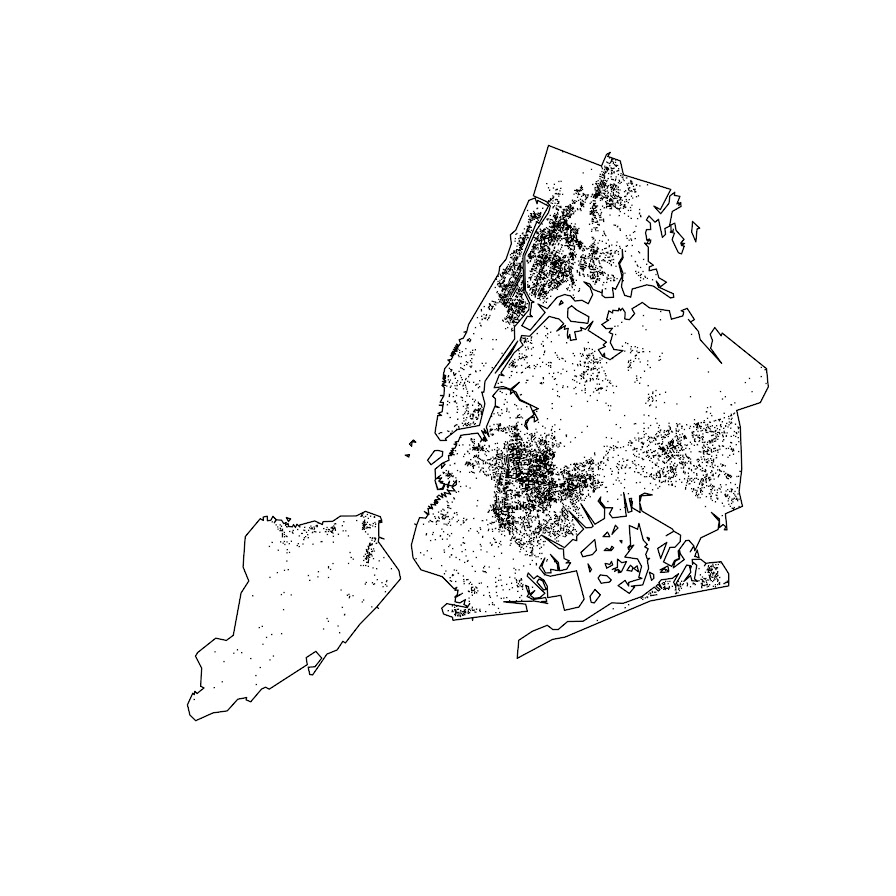The next part I created a function to generate a nice grid over an outline area of your choice to do the SPPT analysis. What this does is generates the regular grid, turns it from a raster to a vector polygon format, and then filters out polygons with 0 overlapping crimes (so in the subsequent SPPT test these areas will all be 0% vs 0%, so not much point in checking them for differences over time!).

You can see the logic from the prior blog post, if I want to use the area with power to detect big changes, I want `N*0.85`. Since I am comparing data over 10 years compared to 1+ years, they are big differences, so I treat N here as 1.5 times the newer dataset, which ends up being around a suggested 3,141 spatial units. Given the area for the overall NYC, this translates to grid cells that are about 1600 by 1600 feet. Once I select out all the 0 grid cells, there only ends up being a total of 1,655 grid cells for the final SPPT analysis.

``````###################################################
# Function to create sppt grid over areas with
# Observed crimes

grid_crimes <- function(outline,crimes,size){
# First creating a raster given the outline extent
base_raster <- raster(ext = extent(outline), res=size)
projection(base_raster) <- crs(outline)
# Getting the coverage for a grid cell over the city area
# Turning into a polygon
base_poly <- rasterToPolygons(base_raster,dissolve=FALSE)
xy_df <- as.data.frame(base_raster,long=T,xy=T)
base_poly\$x <- xy_df\$x
base_poly\$y <- xy_df\$y
base_poly\$poly_id <- 1:nrow(base_poly)
# May also want to select based on layer value
# sel_poly <- base_poly[base_poly\$layer > 0.05,]
# means the grid cell has more than 5% in the outline area
# Selecting only grid cells with an observed crime
ov_crime <- over(crimes,base_poly)
any_crime <- unique(ov_crime\$poly_id)
sub_poly <- base_poly[base_poly\$poly_id %in% any_crime,]
# Redo the id
sub_poly\$poly_id <- 1:nrow(sub_poly)
return(sub_poly)
}

# Calculating suggested sample size
total_counts <- as.data.frame(table(shooting\$Pre))
print(total_counts)

# Lets go with the pre-total times 1.5
total_n <- total_counts\$Freq*1.5

# Figure out the total number of grid cells
# Given the total area
side <- sqrt( total_area/total_n )
print(side)
# 1637, lets just round down to 1600

poly_cells <- grid_crimes(nyc_simpler,shooting,1600)
print(nrow(poly_cells)) #1655

png('NYC_GridCells.png',units='in',res=1000,height=6,width=6,type='cairo')
plot(nyc_simpler)
dev.off()
###################################################
``````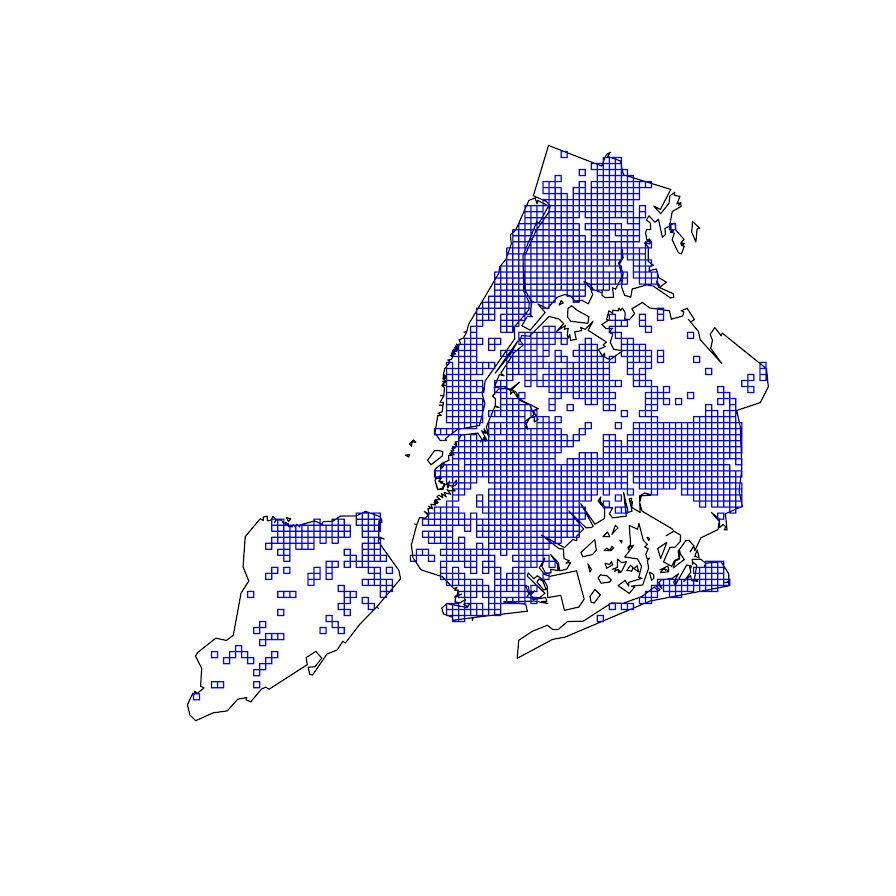Next part is to split the data into pre/post, and do the SPPT analysis. Here I use all the defaults, the Chi-square test for proportional differences, along with a correction for multiple comparisons. Without the multiple comparison correction, we have a total of 174 grid cells that have a p-value < 0.05 for the differences in proportions for an S index of around 89%. With the multiple comparison correction though, the majority of those p-values are adjusted to be above 0.05, and only 25 remain afterwards (98% S-index). You can see in the screenshot that all of those significant differences are increases in proportions from the pre to post. While a few are 0 shootings to a handful of shootings (suggesting diffusion), the majority are areas that had multiple shootings in the historical data, they are just at a higher intensity now.

``````###################################################
# Now lets do the sppt analysis

split_shoot <- split(shooting,shooting\$Pre)
pre <- split_shoot\$`1`
post <- split_shoot\$`0`

library(dplyr)
sppt_diff <- sppt_diff(pre, post, poly_cells)
summary(sppt_diff)

sum(sppt_diff\$p.value < 0.05) #174, around 89% similarity
sum(sppt_diff\$p.adjusted  < 0.05) #25, 98% similarity

# Lets select out the increases/decreases
# And just map those

sppt_sig <- sppt_diff[sig,]
head(sppt_sig,25) # to check out all increases
###################################################
``````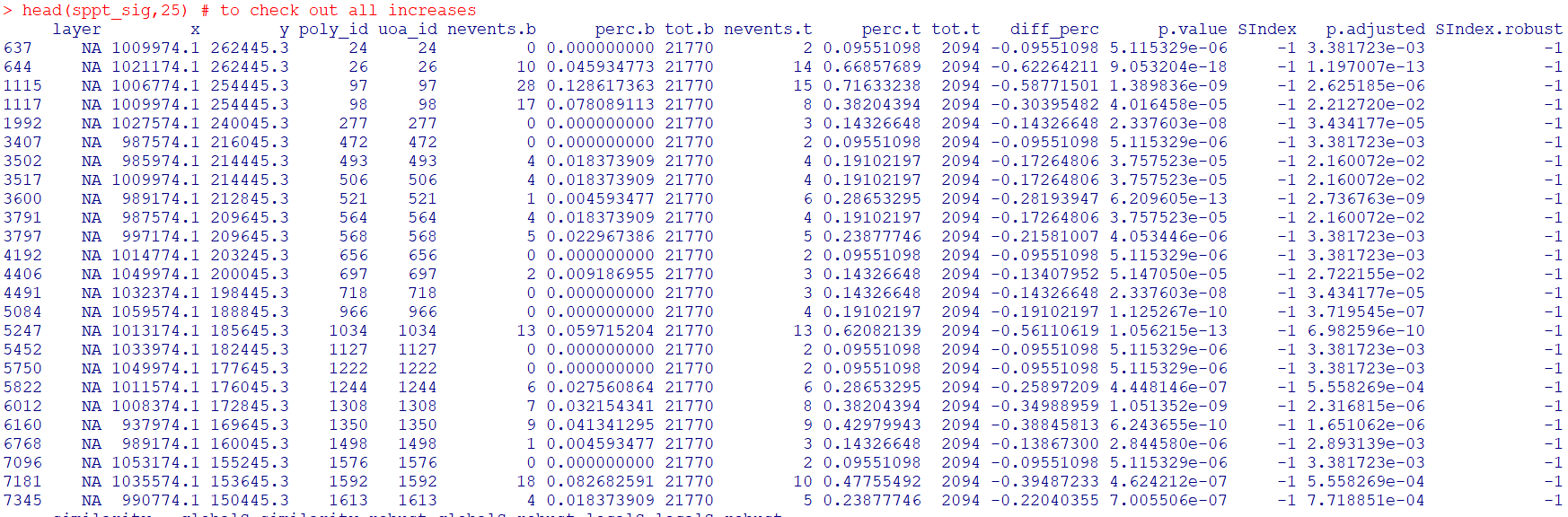The table is not all that helpful though for really digging into patterns, we need to map out the differences. The first here is a map showing the significant grid cells. They are somewhat tiny though, so you have to kind of look close to see where they are. The second map uses proportional circles to the percent difference (so bigger circles show larger increases). I am too lazy to do a legend/scale, but see my prior post on a hexbin map, or the sp website in the comments.

``````###################################################
# Making a map
png('NYC_SigCells.png',units='in',res=1000,height=6,width=6,type='cairo')
plot(nyc_simpler,lwd=1.5)
dev.off()

circ_sizes <- sqrt(-sppt_sig\$diff_perc)*3

png('NYC_SigCircles.png',units='in',res=1000,height=6,width=6,type='cairo')
plot(nyc_simpler,lwd=1.5)
points(coordinates(sppt_sig),pch=21,cex=circ_sizes,bg='red')
dev.off()

# check out https://edzer.github.io/sp/
# For nicer maps/legends/etc.
###################################################
``````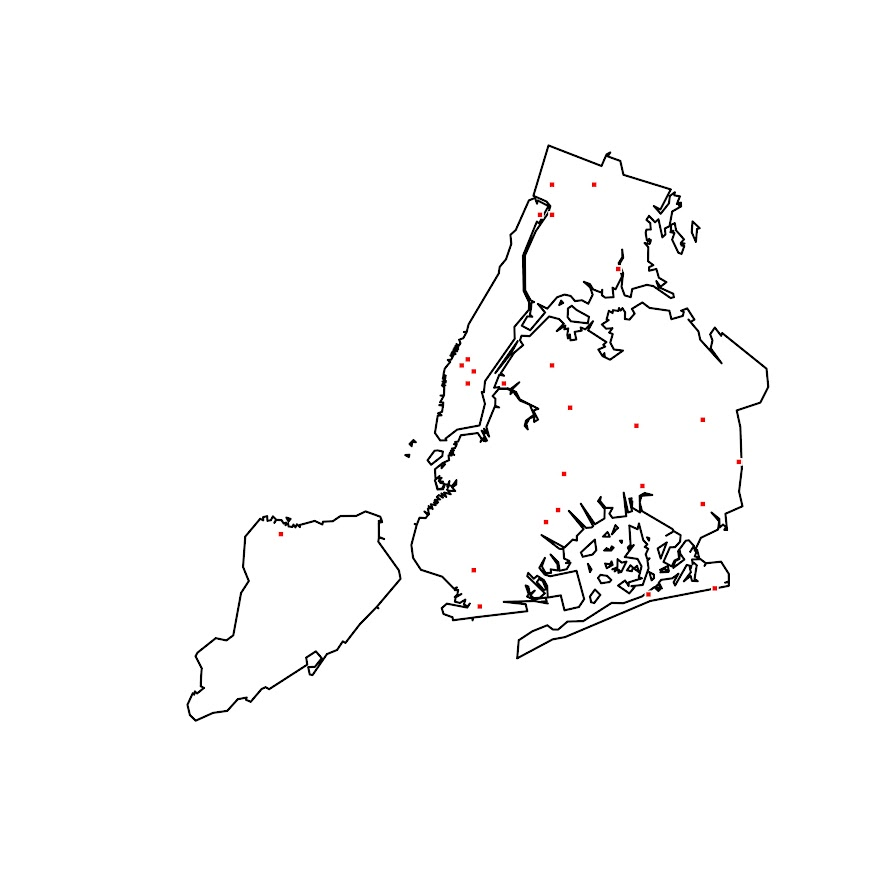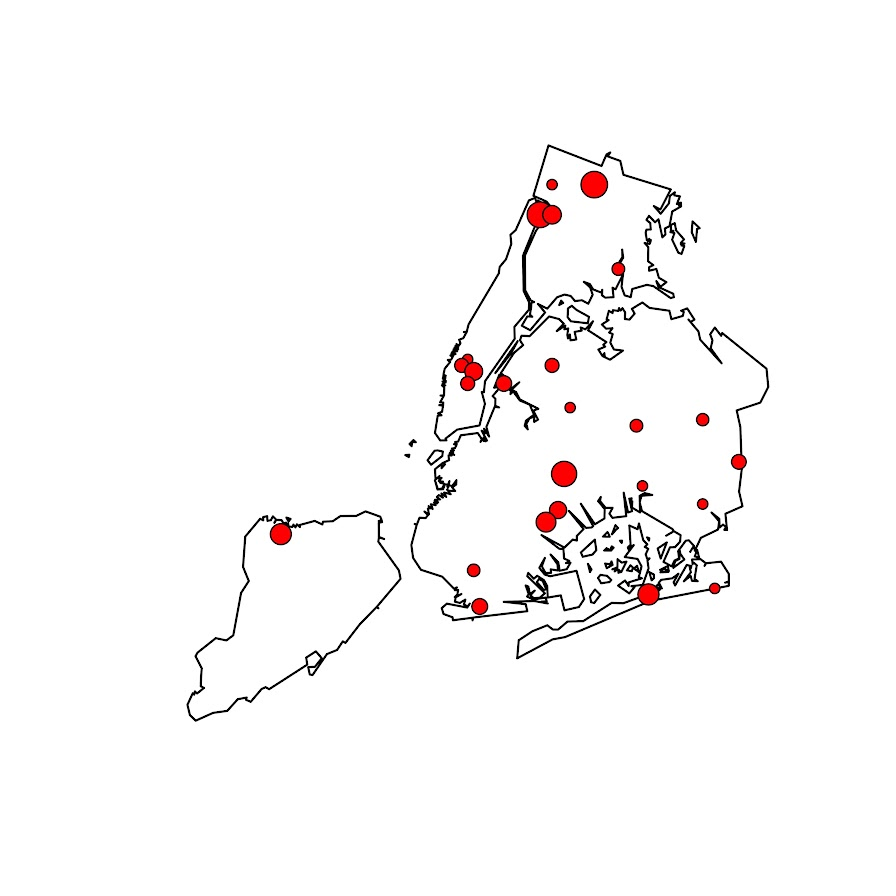So the increases appear pretty spread out. We have a few notable ones that made the news right in the thick of things in Manhattan, but there are examples of grid cells that increased scattered all over the boroughs. I am not going to the trouble here, but if I were a crime analyst working on this, I would export this to a format where I could zoom into the local areas and drill down into the specific incidents. You can do that either in ArcGIS, or more directly in R by creating a leaflet map.

So if folks have any better ideas for testing out crime increases I am all ears. At some point will give the R package sparr a try. (Here you could treat pre as the controls and post as the cases.) I am not a real big fan of over interpreting changes in kernel density estimates though (they can be quite noisy, and heavily influenced by the bandwidth), so I do like the SPPT analysis by default (but it swaps out a different problem with choosing a reasonable grid cell size).

# Spatial sample size suggestions for SPPT analysis

I’ve reviewed several papers recently that use Martin Andresen’s Spatial Point Pattern Test (Andresen, 2016). I have been critical of these papers, as I think they are using too small of samples to be reasonable. So here in this blog post I will lay out spatial sample size recommendations. Or more specifically if you have N crimes, advice about how you can conduct the SPPT test in S spatial units of analysis.

Long story short, if you have N crimes, I think you should either use `0.85*N = S` spatial units of analysis at the high end, but can only detect very large changes. To be well powered to detect smaller changes between the two distributions, use `0.45*N = S`. That is, if you have two crime samples you want to compare, and the smaller sample has 1000 crimes, the largest spatial sample size I would recommend is 850 units, but I think 450 units is better.

For those not familiar with the SPPT technique, it compares the proportion of events falling inside a common area (e.g. police beats, census block groups, etc.) between two patterns. So for example in my work I compared the proportion of violent crime and the proportion of SQF in New York City (Wheeler et al., 2018). I think it makes sense as a gross monitoring metric for PDs this way (say for those doing DDACTS, swap out pedestrian stops with traffic stops), so you can say things like `area A had a much lower proportion of crimes than stops, so we should emphasize people do fewer stops in A overall`.

If you are a PD, you may already know the spatial units you want to use for monitoring purposes (say for each police sector or precinct). In that case, you want the power analysis to help guide you for how large a sample you need to effectively know how often you can update the estimates (e.g. you may only have enough traffic stops and violent crimes to do the estimates on a quarterly basis, not a monthly one) Many academic papers though are just generally theory testing, so don’t have an a priori spatial unit of analysis chosen. (But they do have two samples, e.g. a historical sample of 2000 shootings and a current sample of 1000 shootings.) See Martin’s site for a list of prior papers using the SPPT to see it in action.

I’ve reviewed several papers that examine these proportion changes using the SPPT at very tiny spatial units of analysis, such as street segments. They also happen to have very tiny numbers of overall crimes, and then break the crimes into subsequent subsets. For example reviewed a paper that had around 100 crimes in each subset of interest, and had around 20,000 street segments. I totally get wanting to examine micro place crime patterns – but the SPPT is not well suited for this I am afraid.

Ultimately if you chunk up the total number of crimes into smaller and smaller areas, you will have less statistical power to uncover differences. With very tiny total crime counts, you will be basically only identifying differences between areas that go from 0 to 1 or 0 to 2 etc. It also becomes much more important to control for multiple comparisons when using a large number of spatial units. In general this technique is not going to work out well for micro units of analysis, it will only really work out for larger spatial units IMO. But here I will give my best advice about how small you can reasonably go for the analysis.

# Power analysis logic

There are quite a few different ways people have suggested to determine the spatial sample for areas when conducting quadrat analysis (e.g. when you make your own spatial areas). So one rule of thumb is to use `2*A/N`, where A is the area of the study and N is the total number of events (Paez, 2021).

Using the SPPT test itself, Malleson et al. (2019) identify the area at which the spatial pattern exhibits the highest similarity index with itself using a resampling approach. Ramos et al. (2021) look at the smallest spatial unit at which the crime patterns within that unit show spatial randomness.

So those later two take an error metric based approach (the spatial unit of analysis likely to result in the miminal amount of error, with error defined different ways). I take a different approach here – power analysis. We want to compare to spatial point patterns for proportional differences, how can we construct the test to be reasonably powered to identify differences we want to detect?

I do not have a perfect way to do this power analysis, but here is my logic. Crime patterns are often slightly overdispersed, so here I assume if you split up say 1000 crimes into 600 areas, it will have an NB2 distribution with a mean of `1000/600 = 1.67` and an overdispersion parameter of 2. (I assume this parameter to be 2 for various reasons, based on prior analysis of crime patterns, and that 2 tends to be in the general ballpark for the amount of overdispersion.) So now we want to see what it would take to go from a hot spot of crime, say the 98th percentile of this distribution to the median 50th percentile.

So in R code, to translate the NB2 mean/dispersion to N & P notation results in N & P parameters of `1.0416667` and `0.3846154` respectively:

``````trans_np <- function(mu,disp){
a <- disp
x <- mu^2/(1 - mu + a*mu)
p <- x/(x + mu)
n <- (mu*p)/(1-p)
return(c(n,p))
}

# Mean 1000/600 and dispersion of 2
nb_dis <- trans_np(1000/600,2)``````

Now we want to see what the counts are to go from the 98th to the 50th percentile of this distribution:

``crime_counts <- qnbinom(c(0.98,0.5), size=nb_dis, prob=nb_dis)``

And this gives us a result of ` 8 1` in the `crime_counts` object. So a hot spot place in this scenario will have around 8 crimes, and the median will be around 1 crime in our hypothetical areas. So we can translate these to percentages, and then feed them into R’s `power.prop.test` function:

``````crime_prop <- crime_counts/1000
power.prop.test(n = 1000, p1 = crime_prop, p2 = crime_prop)``````

And this gives us a result of:

``````     Two-sample comparison of proportions power calculation

n = 1000
p1 = 0.008
p2 = 0.001
sig.level = 0.05
power = 0.6477139
alternative = two.sided

NOTE: n is number in *each* group``````

Note that this is for one N estimate, and assumes that N will be the same for each proportion. In practice for the SPPT test this is not true, oftentimes we have two crime samples (or crime vs police actions like stops), which have very different total baseline N’s. (It is part of the reason the test is useful, it doesn’t make so much sense in that case to compare densities as it does proportions.) So subsequently when we do these estimates, we should either take the average of the total number of crimes we have in our two point patterns for SPPT (if they are close to the same size), or the minimum number of events if they are very disparate. So if you have in sample A 1000 crimes, and sample B 2000 crimes, I think you should treat the N in this scenario as 1500. If you have 5000 crimes vs 1000000 crimes, you should treat N here as 5000.

So that estimate above is for one set of crimes (1000), and one set of areas (600). But what if we vary the number of areas? At what number of areas do we have the maximum power?

So I provide functions below to generate the power estimate curve, given these assumptions about the underlying crime distribution (which will generally be in the ballpark for many crime patterns, but not perfect), for varying numbers of spatial units. Typically we know the total number of crimes, so we are saying given I have N crimes, how finely can a split them up to check for differences with the SPPT test.

Both the Malleson and Ramos article place their recommendations in terms of area instead of total number of units. But it would not surprise me if our different procedures end up resulting in similar recommendations based on the observed outputs of each of the papers. (The `2A/N` quadrat analysis suggestion translates to `N*0.5` total number of areas, pretty close to my `0.45*N` suggestion for example.)

# R Code

Below I have a nicer function to do the analysis I walked through above, but give a nice power curve and dataframe over various potential spatial sample sizes:

``````# SPPT Power analysis example
library(ggplot)

# See https://andrewpwheeler.com/2015/01/03/translating-between-the-dispersion-term-in-a-negative-binomial-regression-and-random-variables-in-spss/
trans_np <- function(mu,disp){
a <- disp
x <- mu^2/(1 - mu + a*mu)
p <- x/(x + mu)
n <- (mu*p)/(1-p)
return(c(n,p))
}

diff_suggest <- function(total_crimes,areas=round(seq(2,total_crimes*10,length.out=500)),
change_quant=c(0.98,0.5), nb_disp=2, alpha = 0.05,
plot=TRUE){
# Figure out mean
areas <- unique(areas)
mean_cr <- total_crimes/areas
# Initialize some vectors to place the results
n_areas <- length(areas)
power <- vector("numeric",length=n_areas)
hign <- power
lown <- power
higp <- power
lowp <- power
# loop over areas and calculate power
for (i in 1:length(areas)){
# Negative binomial parameters
dp <- trans_np(mean_cr[i],nb_disp)
hilo <- qnbinom(change_quant, size=dp, prob=dp)
hilo_prop <- hilo/total_crimes
# Power for test
pow <- power.prop.test(n = total_crimes, p1 = hilo_prop, p2 = hilo_prop,
sig.level = alpha)
# Stuffing results in vector
power[i] <- pow\$power
hign[i] <- hilo
lown[i] <- hilo
higp[i] <- hilo_prop
lowp[i] <- hilo_prop
}
Ncrimes <- rep(total_crimes,n_areas)
res_df <- data.frame(Ncrimes,areas,mean_cr,power,hign,lown,higp,lowp)
# replacing missing with 0
res_df[is.na(res_df)] <- 0
if (plot) {
require(ggplot2)
fmt_cr <- formatC(total_crimes, format="f", big.mark=",", digits=0)
title_str <- paste0("Power per area for Total number of crimes: ",fmt_cr)
cap_str <- paste0("NB Dispersion = ",nb_disp,", alpha = ",alpha,
", change quantiles = ",change_quant," to ",change_quant)
p <- ggplot(data=res_df,aes(x=areas,y=power)) + geom_line(size=1.5) +
theme_bw() + theme(panel.grid.major = element_line(linetype="dashed")) +
labs(x='Number of Areas',y=NULL,title=title_str,caption=cap_str) +
scale_x_continuous(minor_breaks=NULL) + scale_y_continuous(minor_breaks=NULL) +
theme(text = element_text(size=16), axis.title.y=element_text(margin=margin(0,10,0,0)))
print(p)
}
return(res_df)
}``````

Once that function is defined, you can make a simple call like below, and it gives you a nice graph of the power given different numbers of grid cells:

``diff_suggest(100)``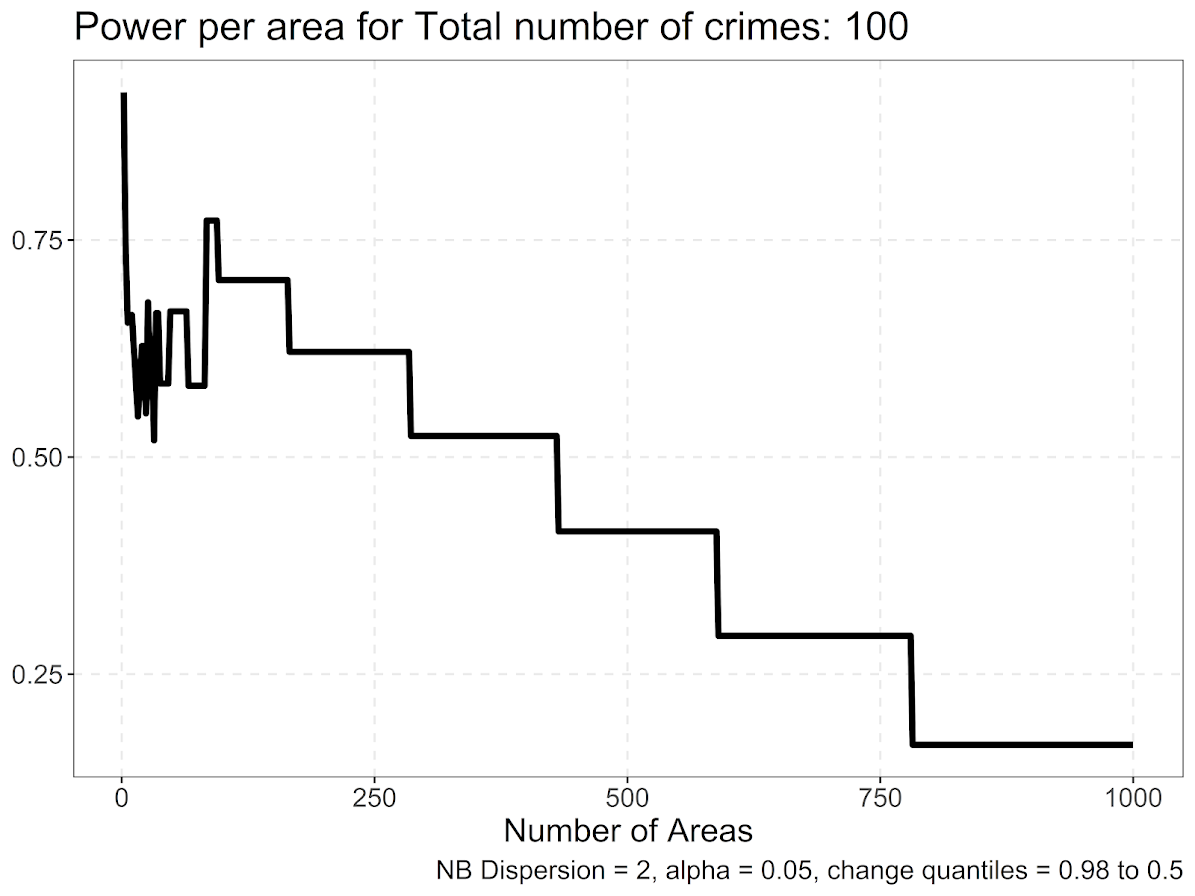So you can see here we never have very high power over this set of parameters. It is also non-monotonic and volatile at very small numbers of spatial units of analysis (at which the overdispersion assumption likely does not hold, and probably is not of much interest). But once that volatility tamps down we have stepped curve, that ends up happening to step whenever the original NB distribution changes from particular integer values.

So what happens with the power curve if we up the number of crimes to 3000?

``diff_suggest(3000)``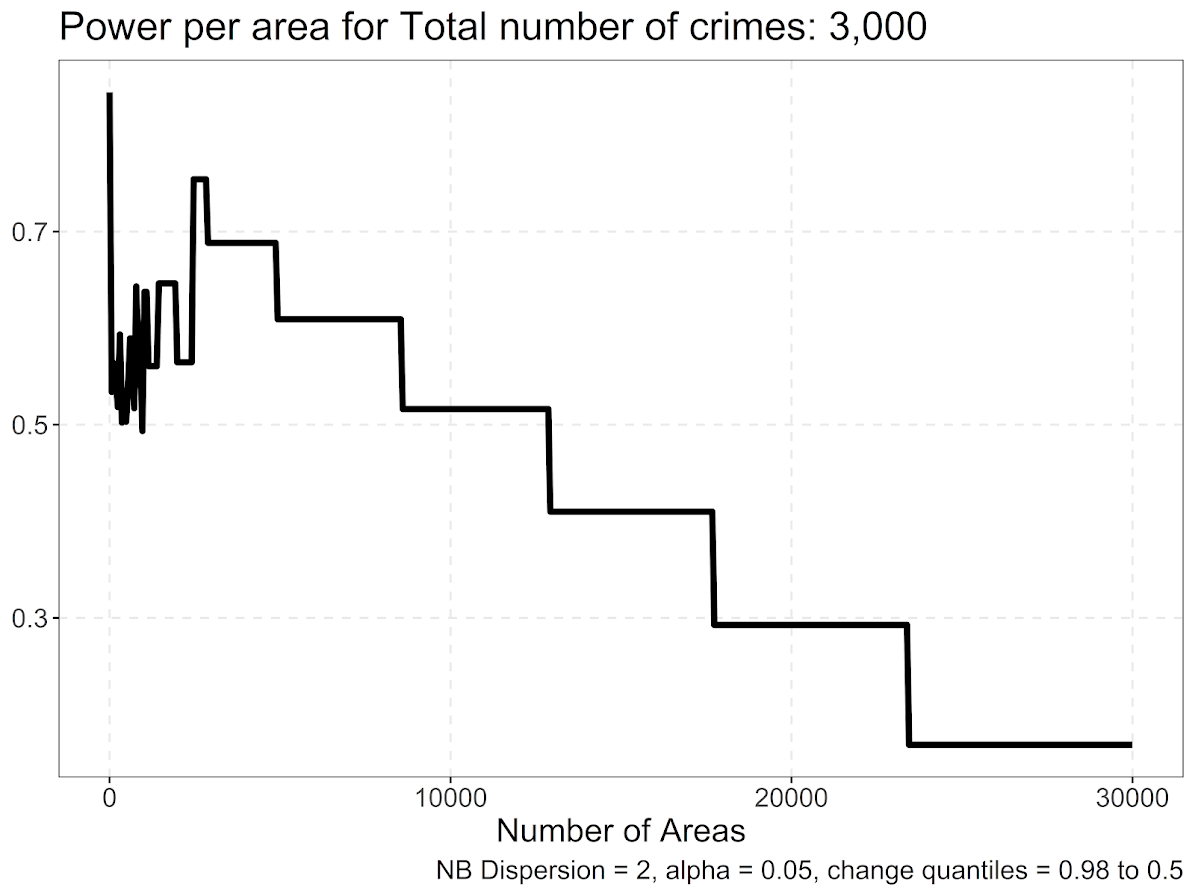So those two patterns are quite similar. It happens that when breaking down to the smaller units, the highest power scenario is when the crimes are subdivided into around 0.85 fewer spatial units than total crimes. So if you have 1000 crimes, in this scenario I would suggest to use 850 areas.

Also note the behavior when you break it down into a very large number of spatial units S, where `S >> N`, you get a progressive decline until around 0 power in this analysis. E.g. if you have 100 times more spatial units than observations, only a handful of locations have any crimes, and the rest are all 0’s. So you need to be able to tell the difference between 1/N and 0, which is tough (and any inferences you do make will just pretty much be indistinguishable from noise).

What about if we change the quantiles we are examining, and instead of looking at the very high crime place to the median, look at the 80th percentile to the 20th percentile:

``diff_suggest(3000, change_quant=c(0.8,0.2))``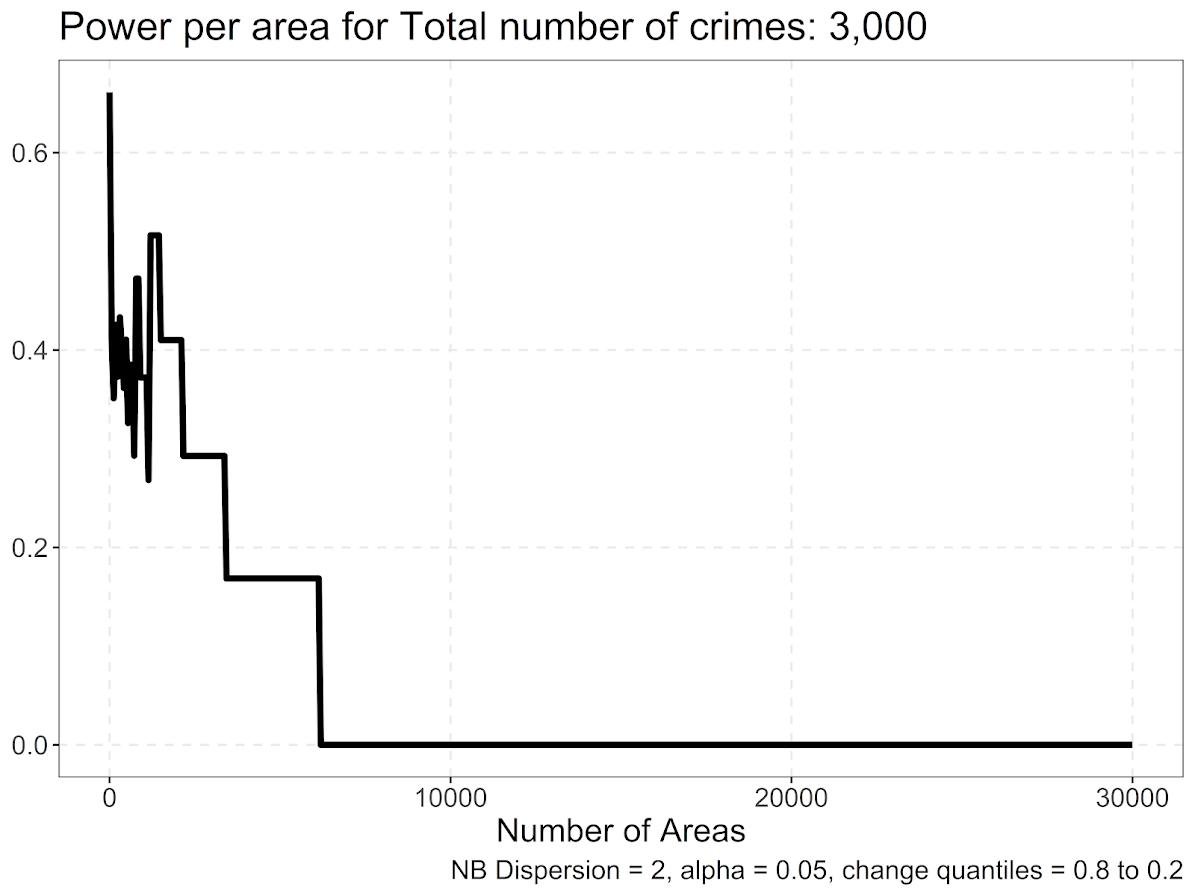We have a similar step pattern, but here the power is never as high as before, and only maxes out slightly above 0.5. It happens that this 0.5 power is around `number of crimes*0.45`. So this suggests that to uncover more middling transitions, one would need to have less than half the number of spatial units of crime observed. E.g. if you have 1000 crimes, I would not suggest any more than 450 spatial units of analysis.

So the first scenario, `crimes*0.85` you could say something like this is the highest power scenario to detect changes from very high crime locations (aka hot spots), to the middle of the distribution. For the second scenario (my preferred offhand), is to say `crimes*0.45` total spatial units results in the highest power scenario to detect more mild changes in the middle of the distribution (and detecting changes from hot spots to cold spots thus have even more power).

For now that is the best advice I can give for determining the spatial sample size for the SPPT test. Will have a follow up blog post on using R to make a grid to conduct the test.

Also I have been wondering about the best way to quantify changes in the overall ranking. I have not come upon a great solution I am happy with though, so will need to think about it some more.

# CCTV and clearance rates paper published

My paper with Yeondae Jung, The effect of public surveillance cameras on crime clearance rates, has recently been published in the Journal of Experimental Criminology. Here is a link to the journal version to download the PDF if you have access, and here is a link to an open read access version.

The paper examines the increase in case clearances (almost always arrests in this sample) for incidents that occurred nearby 329 public CCTV cameras installed and monitored by the Dallas PD from 2014-2017. Quite a bit of the criminological research on CCTV cameras has examined crime reductions after CCTV installations, which the outcome of that is a consistent small decrease in crimes. Cameras are often argued to help solve cases though, e.g. catch the guy in the act. So we examined that in the Dallas data.

We did find evidence that CCTV increases case clearances on average, here is the graph showing the estimated clearances before the cameras were installed (based on the distance between the crime location and the camera), and the line after. You can see the bump up for the post period, around 2% in this graph and tapering off to an estimate of no differences before 1000 feet.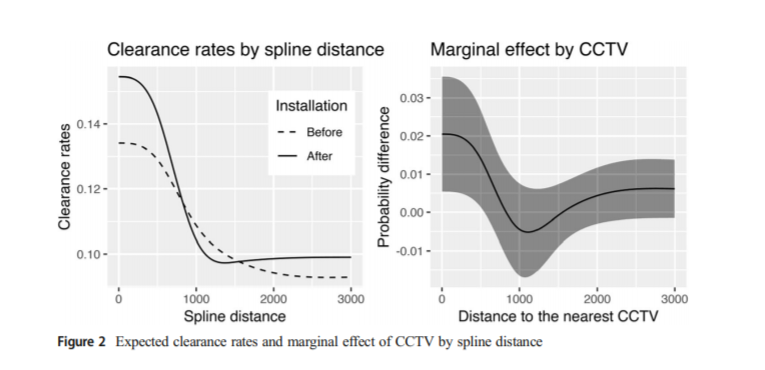When we break this down by different crimes though, we find that the increase in clearances is mostly limited to theft cases. Also we estimate counterfactual how many extra clearances the cameras were likely to cause. So based on our model, we can say something like, a case would have an estimated probability of clearance without a camera of 10%, but with a camera of 12%. We can then do that counterfactual for many of the events around cameras, e.g.:

``````Probability No Camera   Probability Camera   Difference
0.10                      0.12             + 0.02
0.05                      0.06             + 0.01
0.04                      0.10             + 0.06``````

And in this example for the three events, we calculate the cameras increased the total expected number of clearances to be `0.02 + 0.01 + 0.06 = 0.09`. This marginal benefit changes for crimes mostly depends on the distance to the camera, but can also change based on when the crime was reported and some other covariates.

We do this exercise for all thefts nearby cameras post installation (over 15,000 in the Dallas data), and then get this estimate of the cumulative number of extra theft clearances we attribute to CCTV: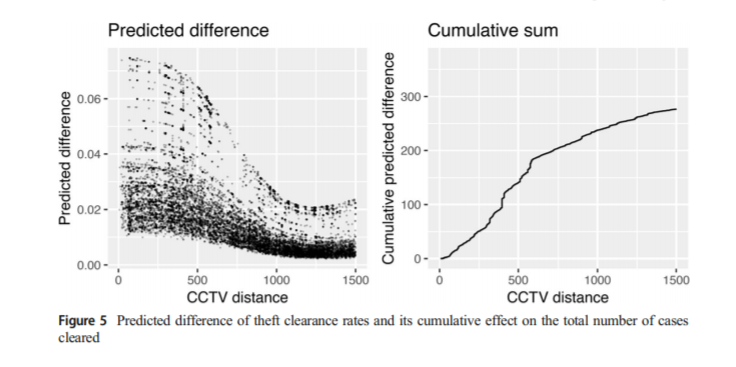So even with 329 cameras and over a year post data, we only estimate cameras resulted in fewer than 300 additional theft clearances. So there is unlikely any reasonable cost-benefit analysis that would suggest cameras are worthwhile for their benefit in clearing additional cases in Dallas.

For those without access to journals, we have the pre-print posted here. The analysis was not edited any from pre-print to published, just some front end and discussion sections were lightly edited over the drafts. Not sure why, but this pre-print is likely my most downloaded paper (over 4k downloads at this point) – even in the good journals when I publish a paper I typically do not get 1000 downloads.

To go on, complaint number 5631 about peer review – this took quite a while to publish because it was rejected on R&R from Justice Quarterly, and with me and Yeondae both having outside of academia jobs it took us a while to do revisions and resubmit. I am not sure the overall prevalence of rejects on R&R’s, I have quite a few of them though in my career (4 that I can remember). The dreaded send to new reviewers is pretty much guaranteed to result in a reject (pretty much asking to roll a Yahtzee to get it past so many people).

We then submitted to a lower journal, The American Journal of Criminal Justice, where we had reviewers who are not familiar with what counterfactuals are. (An irony of trying to go to a lower journal for an easier time, they tend to have much worse reviewers, so can sometimes be not easier at all.) I picked it up again a few months ago, and re-reading it thought it was too good to drop, and resubmitted to the Journal of Experimental Criminology, where the reviews were reasonable and quick, and Wesley Jennings made fast decisions as well.

# Some microsynth notes

My question is from our CPP project on business improvement districts (Piza, Wheeler, Connealy, Feng 2020). The article indicates that you ran three of the microsynth matching variables as an average over each instead of the cumulative sum (street length, percent new housing structures, percent occupied structures). How did you get R to read the variables as averages instead of the entire sum of the treatment period of interest? I have the microsynth code you used to generate our models, but cannot seem to determine how you got R to read the variables as averages.

So Nate is talking about this paper, Crime control effects of a police substation within a business improvement district: A quasi-experimental synthetic control evaluation (Piza et al., 2020), and here is the balance table in the paper: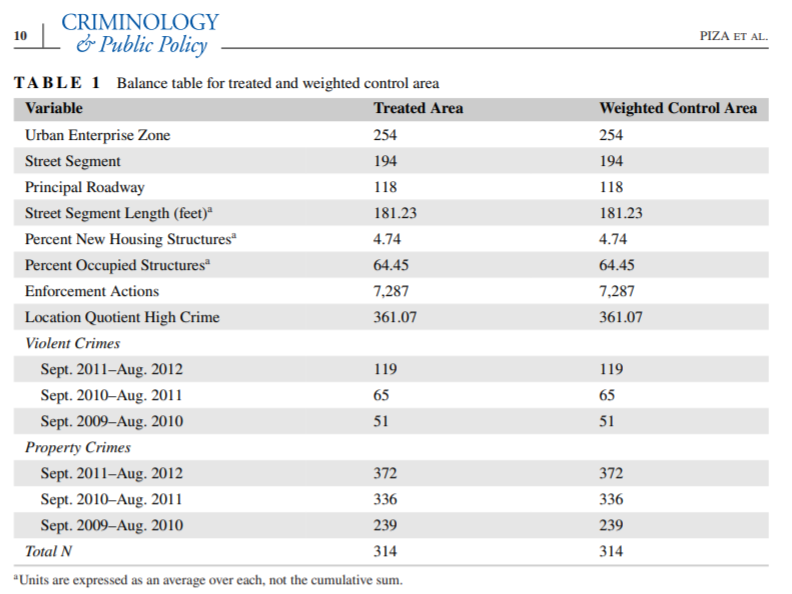To be clear to folks, I did not balance on the averages, but simply reported the table in terms of averages. So here is the original readout from R: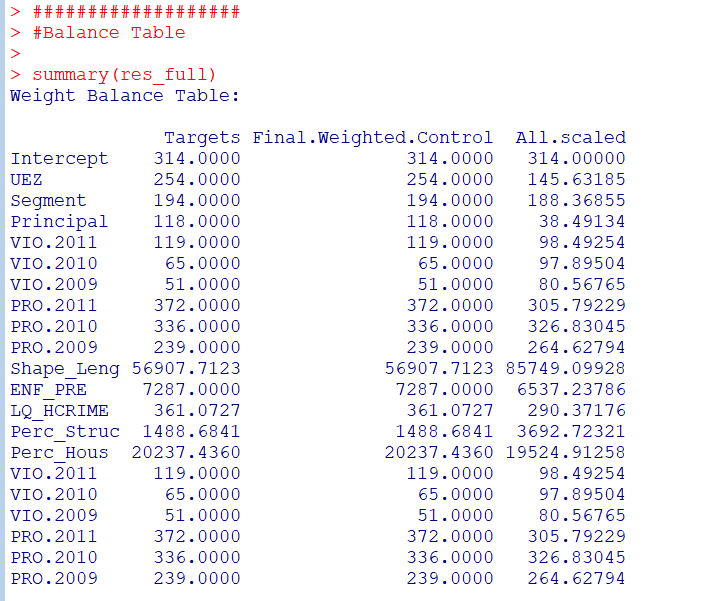So I just divided those noted rows by 314 to make them easier to read. You could divide values by the total number of treated units though in the original data to have microsynth match on the averages instead if you wanted to. Example below (this is R code, see the microsynth library and paper by Robbins et al., 2017):

``````library(microsynth)
set.seed(10)

data(seattledmi) #just using data in the package
cs <- seattledmi
# calculating proportions
cs\$BlackPerc <- (cs\$BLACK/cs\$TotalPop)*100
cs\$FHHPerc <- (cs\$FEMALE_HOU/cs\$HOUSEHOLDS)*100
# replacing 0 pop with 0
cs[is.na(cs)] <- 0

cov.var <- c("TotalPop","HISPANIC","Males_1521","FHHPerc","BlackPerc")
match.out <- c("i_felony", "i_misdemea")

sea_prop <- microsynth(cs,
idvar="ID", timevar="time", intvar="Intervention",
start.pre=1, end.pre=12, end.post=16,
match.out.min=match.out,match.out=FALSE,
match.covar=FALSE,check.feas=FALSE,
match.covar.min=cov.var,
result.var=match.out)

summary(sea_prop) # balance table``````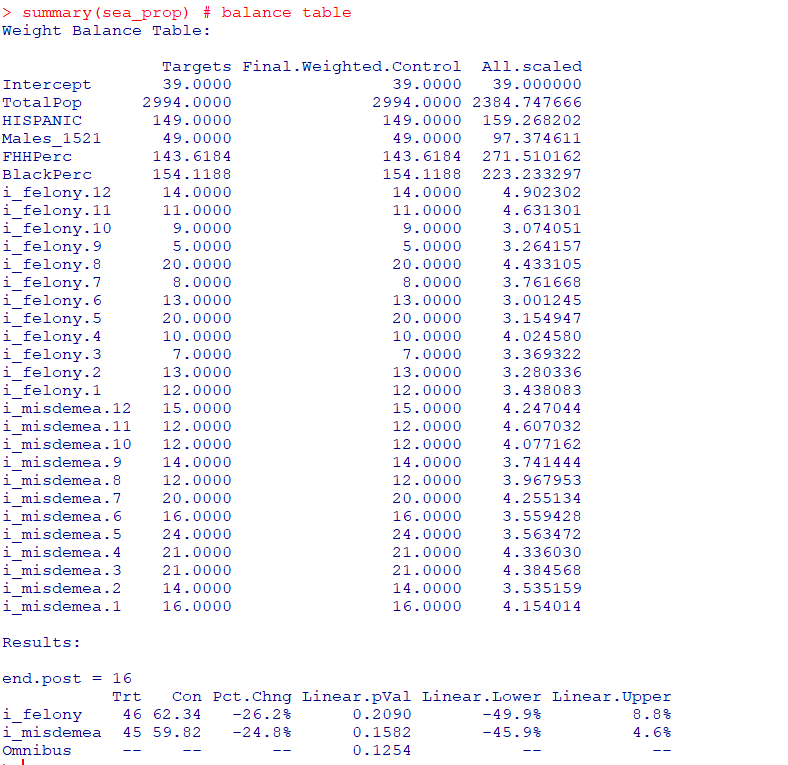And here you can see that we are matching on the cumulative sums for each of the areas, but we can divide our covariates by the number of treated units, and we will match on the proportional values.

``````# Can divide by 39 and get the same results
cs[,cov.var] <- cs[,cov.var]/39

sea_div <- microsynth(cs,
idvar="ID", timevar="time", intvar="Intervention",
start.pre=1, end.pre=12, end.post=16,
match.out.min=match.out,match.out=FALSE,
match.covar=FALSE,check.feas=FALSE,
match.covar.min=cov.var,
result.var=match.out)

summary(sea_div) # balance table``````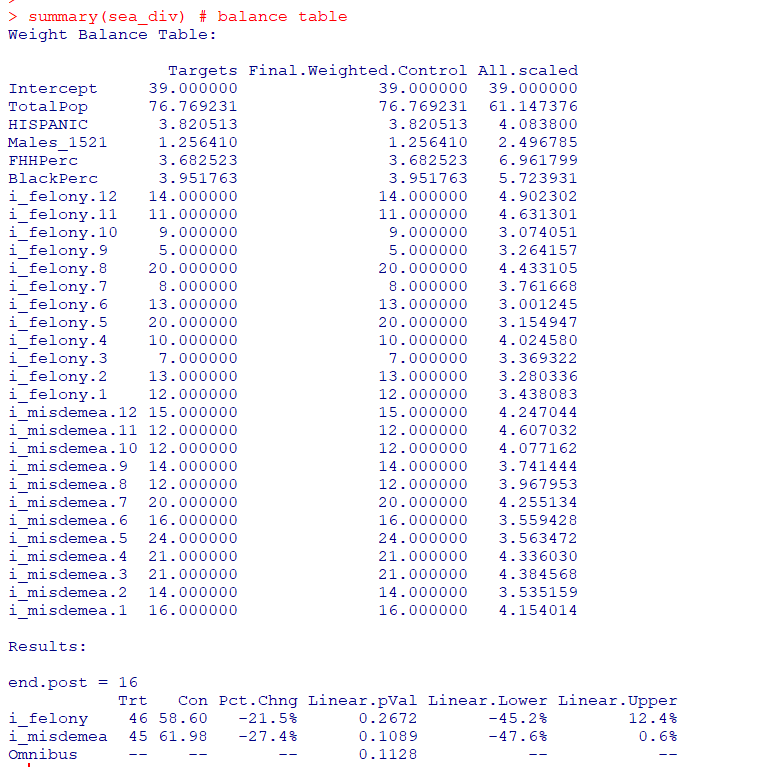Note that these do not result in the same weights. If you look at the results you will see the treatment effects are slightly different. Also if you do:

``````# Showing weights are not equal
all.equal(sea_div\$w\$Weights,sea_prop\$w\$Weights)``````

It does not return True. Honestly not familiar enough with the procedure that microsynth uses to do the matching (Raking survey weights) to know if this is due to stochastic stuff or due to how the weighting algorithm works (I would have thought a linear change does not make a difference, but I was wrong).

On the bucket list is to do a matching algorithm that returns geographically contiguous areas and gives the weights all values of 1 (so creates comparable neighborhoods), instead of estimating Raking weights. That may be 5 years though before I get around to that. Gio has a nice map to show the way the weights work now is they may be all over the place (Circo et al., 2021) – I am not sure that is a good thing though.

But I did want to share some functions I used for the paper I worked with Nate on. First, this is for if you use the permutation approach, the function `prep_synth` returns some of the data in a nicer format to make graphs and calculate your own stats:

``````# Function to scoop up the data nicely
prep_synth <- function(mod){
#Grab the plot data
plotStats <- mod[['Plot.Stats']]
#For the left graph
Treat <- as.data.frame(t(plotStats\$Treatment))
Treat\$Type <- "Treat"
#This works for my data at years, will not
#Be right for data with more granular time though
Treat\$Year <- as.integer(rownames(Treat))
Cont <- as.data.frame(t(plotStats\$Control))
Cont\$Type <- "Control"
Cont\$Year <- as.integer(rownames(Cont))
AllRes <- rbind(Treat,Cont)
#For the right graph
Perm <- as.data.frame(t(as.data.frame(plotStats\$Difference)))
SplitStr <- t(as.data.frame(strsplit(rownames(Perm),"[.]")))
colnames(SplitStr) <- c("Type","Year")
rownames(SplitStr) <- 1:nrow(SplitStr)
SplitStr <- as.data.frame(SplitStr)
Perm\$Type <- as.character(SplitStr\$Type)
Perm\$Year <- as.integer(as.character(SplitStr\$Year))
Perm\$Group <- ifelse(Perm\$Type == 'Main','Treatment Effect','Permutations')
#Reordering factor levels for plots
AllRes\$Type <- factor(AllRes\$Type,levels=c('Treat','Control'))
levels(AllRes\$Type) <- c('Treated','Synthetic Control')
Perm\$Group <- factor(Perm\$Group,levels=c('Treatment Effect','Permutations'))
#Exporting result
Res <- vector("list",length=2)
Res[] <- AllRes
Res[] <- Perm
names(Res) <- c("AggOutcomes","DiffPerms")
return(Res)
}``````

It works for the prior tables, but I really made these functions to work with when you used permutations to get the errors. (In the micro synth example, it is easier to work with permutations than in the state level example for synth, in which I think conformal prediction intervals makes more sense, see De Biasi & Circo, 2021 for a recent real example with micro place based data though.)

``````# Takes like 1.5 minutes
sea_perm <- microsynth(seattledmi,
idvar="ID", timevar="time", intvar="Intervention",
start.pre=1, end.pre=12, end.post=16,
match.out.min=match.out,match.out=FALSE,
match.covar=FALSE,check.feas=FALSE,
match.covar.min=cov.var,
result.var=match.out, perm=99)

res_prop <- prep_synth(sea_perm)
print(res_prop)``````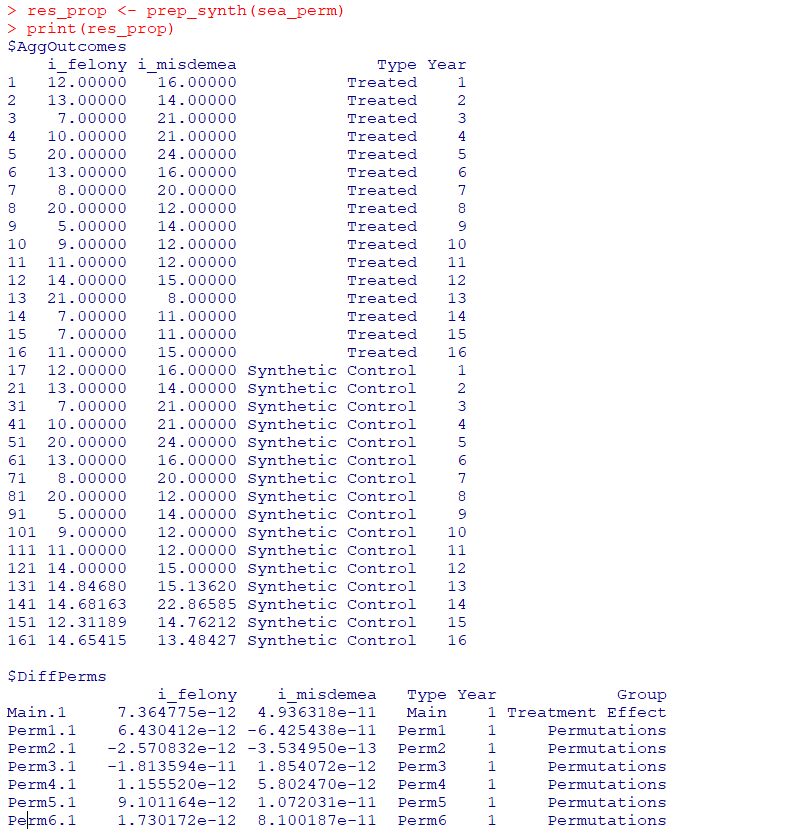So the dataframe in the first slot is the overall treatment effect, and the second dataframe is a nice stacked version for the permutations. First, I really do not like the percentage change (see Wheeler, 2016 for the most direct critique, but I have a bunch on this site). So I wrote code to translate the treatment effects into crime count reductions instead of the percent change stuff.

``````# Getting the observed treatment effect on count scale
# vs the permutations

agg_fun <- function(x){
sdx <- sd(x)
minval <- min(x)
l_025 <- quantile(x, probs=0.025)
u_975 <- quantile(x, probs=0.975)
maxval <- max(x)
totn <- length(x)
res <- c(sdx,minval,l_025,u_975,maxval,totn)
return(res)
}

treat_count <- function(rp){
# Calculating the treatment effect based on permutations
keep_vars <- !( names(rp[]) %in% c("Year","Group") )
out_names <- names(rp[])[keep_vars][1:(sum(keep_vars)-1)]
loc_dat <- rp[][,keep_vars]
agg_treat <- aggregate(. ~ Type, data = loc_dat, FUN=sum)
n_cols <- 2:dim(agg_treat)
n_rows <- 2:nrow(agg_treat)
dif <- agg_treat[rep(1,max(n_rows)-1),n_cols] - agg_treat[n_rows,n_cols]
dif\$Const <- 1
stats <- aggregate(. ~ Const, data = dif, FUN=agg_fun)
v_names <- c("se","min","low025","up975","max","totperm")
long_stats <- reshape(stats,direction='long',idvar = "Const",
varying=list(2:ncol(stats)),
v.names=v_names, times=out_names)
# Add back in the original stats
long_stats <- long_stats[,v_names]
rownames(long_stats) <- 1:nrow(long_stats)
long_stats\$observed <- t(agg_treat[1,n_cols])[,1]
long_stats\$outcome <- out_names
ord_vars <- c('outcome','observed',v_names)
return(long_stats[,ord_vars])
}

treat_count(res_prop)``````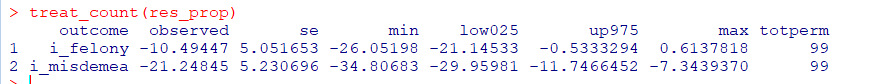So that is the cumulative total effect of the intervention. This is more similar to the WDD test (Wheeler & Ratcliffe, 2018), but since the pre-time period is matched perfectly, just is the differences in the post time periods. And here it uses the permutations to estimate the error, not any Poisson approximation.

But I often see folks concerned about the effects further out in time for synthetic control studies. So here is a graph that just looks at the instant effects for each time period, showing the difference via the permutation lines:

``````# GGPLOT graphs, individual lines
library(ggplot2)
perm_data <- res_prop[]
# Ordering factors to get the treated line on top
perm_data\$Group <- factor(perm_data\$Group, c("Permutations","Treatment Effect"))
perm_data\$Type <- factor(perm_data\$Type, rev(unique(perm_data\$Type)))
pro_perm <- ggplot(data=perm_data,aes(x=Year,y=i_felony,group=Type,color=Group,size=Group)) +
geom_line() +
scale_color_manual(values=c('grey','red')) + scale_size_manual(values=c(0.5,2)) +
geom_vline(xintercept=12) + theme_bw() +
labs(x=NULL,y='Felony Difference from Control') +
scale_x_continuous(minor_breaks=NULL, breaks=1:16) +
scale_y_continuous(breaks=seq(-10,10,2), minor_breaks=NULL) +
theme(panel.grid.major = element_line(linetype="dashed"), legend.title= element_blank(),
legend.position = c(0.2,0.8), legend.background = element_rect(linetype="solid", color="black")) +
theme(text = element_text(size=16), axis.title.y=element_text(margin=margin(0,10,0,0)))``````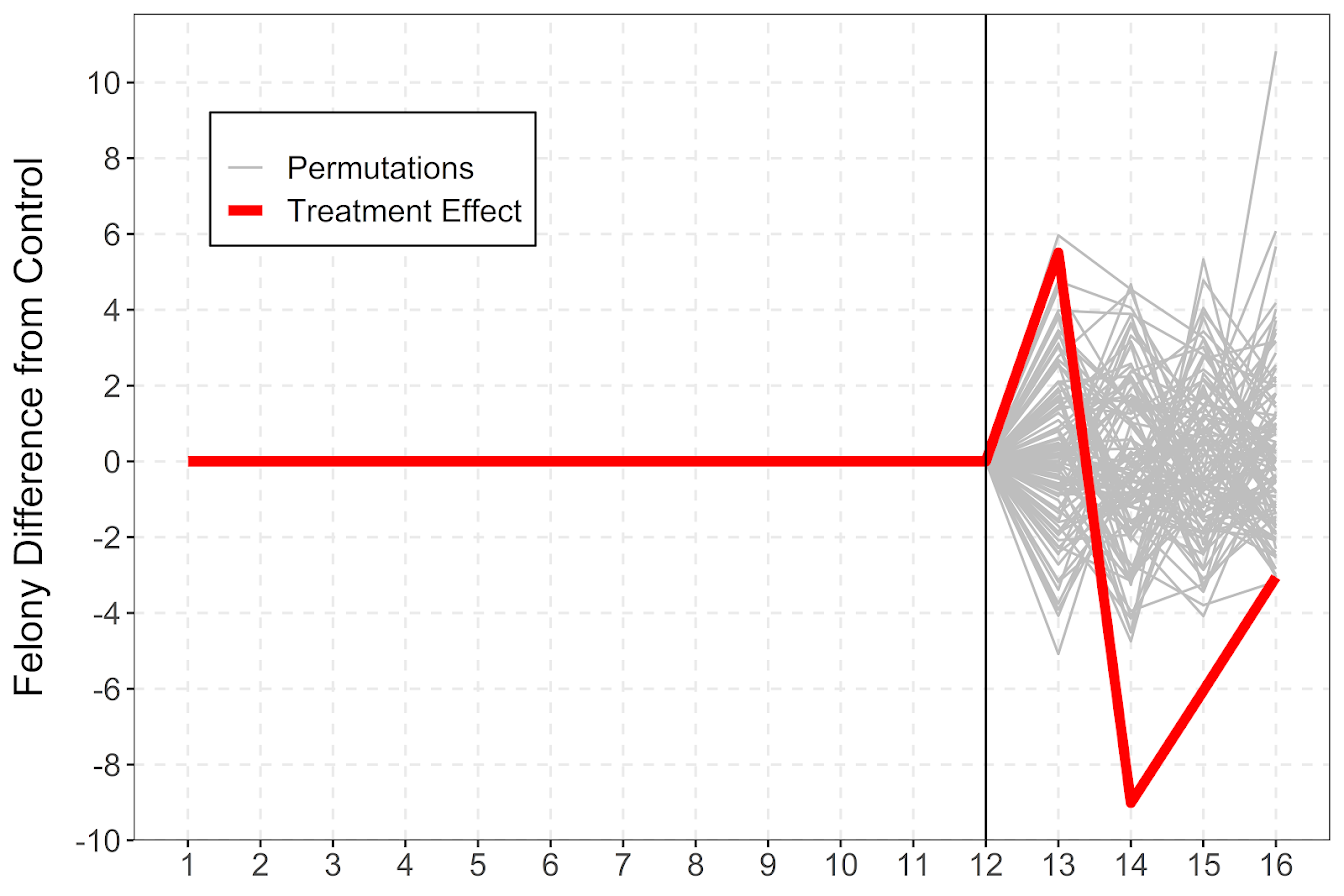And I also like looking at this for the cumulative effects as well, which you can see with the permutation lines widen over time.

``````# Cumulative vs Pointwise
perm_data\$csum_felony <- ave(perm_data\$i_felony, perm_data\$Type, FUN=cumsum)
pro_cum  <- ggplot(data=perm_data,aes(x=Year,y=csum_felony,group=Type,color=Group,size=Group)) +
geom_line() +
scale_color_manual(values=c('grey','red')) + scale_size_manual(values=c(0.5,2)) +
geom_vline(xintercept=12) + theme_bw() +
labs(x=NULL,y='Felony Difference from Control Cumulative') +
scale_x_continuous(minor_breaks=NULL, breaks=1:16) +
scale_y_continuous(breaks=seq(-20,20,5), minor_breaks=NULL) +
theme(panel.grid.major = element_line(linetype="dashed"), legend.title= element_blank(),
legend.position = c(0.2,0.8), legend.background = element_rect(linetype="solid", color="black")) +
theme(text = element_text(size=16), axis.title.y=element_text(margin=margin(0,10,0,0)))``````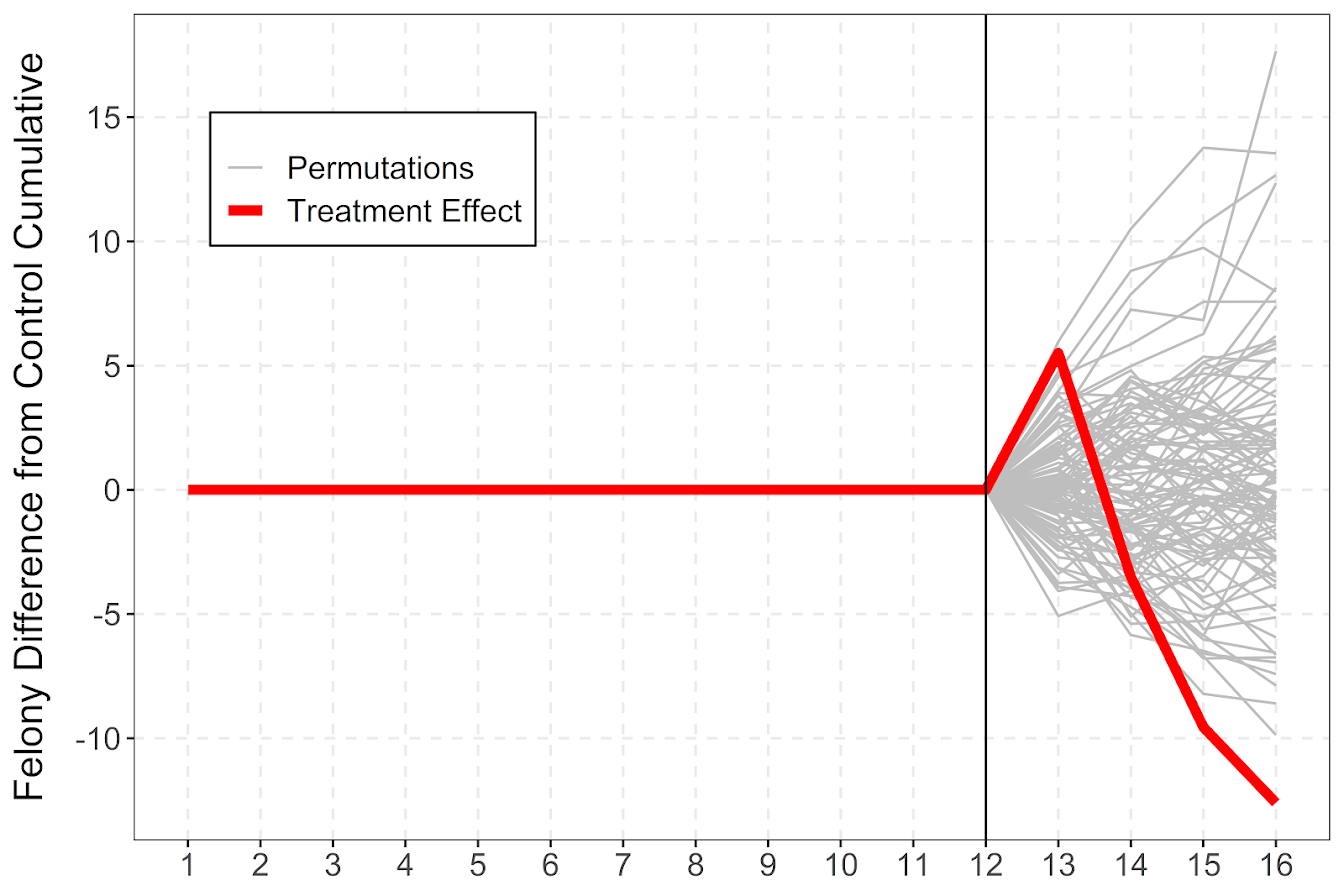If you do a ton of permutations (say 999 instead of 99), it would likely make more sense to do a fan chart type error bars and show areas of different percentiles instead of each individual line (Yim et al., 2020).

I will need to slate a totally different blog post to discuss instant vs cumulative effects for time series analysis. Been peer-reviewing quite a few time series analyses of Covid and crime changes – most everyone only focuses on instant changes, and does not calculate cumulative changes. See for example estimating excess deaths for the Texas winter storm power outage (Aldhous et al., 2021). Folks could do similar analyses for short term crime interventions. Jerry has a good example of using the Causal Impact package to estimate cumulative effects for a gang takedown intervention (Ratcliffe et al., 2017) for one criminal justice example I am familiar with.

Again for folks feel free to ask me anything. I may not always be able to do as deep a dive as this, but always feel free to reach out.

# Open source code projects in criminology

As part of my work with CrimRxiv, we have started the idea of creating a page to link to various open source criminology focused projects. That is overly broad, but high level here we are thinking for pragmatic resources (e.g. code repositories/packages, open source text books), as opposed to more traditional literature.

As part of our overlay journal we are starting, D1G1TAL & C0MPUTAT10NAL CR1M1N0L0GY, we are trying to get folks to submit open source work for a paper. (As a note, this will not have any charges to publish.) The motivation is two-fold: 1) this gives a venue to get your code peer reviewed (e.g. similar to the Journal of Open Source Software). This is mainly for the writer, to give academic recognition for your open source work. 2) Is for the consumer of the information, it is a nice place to keep up on current developments. If you write an R package to do some cool analysis I want to be aware of it!

For 2, we can accomplish something similar by just linking to current projects. I have started a spreadsheet of links I am collating for now, (in the future will update to this page, you need to be signed into CrimRxiv to see that list). For examples of the work I have collated so far:

Then we have various R packages from folks floating around; Greg Ridgeway, Jerry Ratcliffe, Wouter Steenbeek (as well as the others I mentioned previously you can check out their other projects on Github). Please add in info into the google spreadsheet, comment here, or send me an email if you would like some work you have done (or know others have done) that should be added.

# Down the rabbit hole with R functions

I had a friend the other day ask me about modifying the plot that goes with R’s boxCox function. In particular they had multiple plots, and wanted to make the Y axes consistent between the different dependent variables. So for a typical R base plot call, you can specify `ylim = c(whatever_low, whatever_high)`, but if you look at function in the end it does not let you do this yourself (it fixes ylim based on the log-likelihood range.

``````library(car)
data(trees)
# Making a second Y variable for illustration later
trees\$V2 <- trees\$Volume*2 + 3*rnorm(nrow(trees))

# Original function, https://rdrr.io/rforge/car/man/boxCox.html
orig_output <- with(trees, boxCox(Volume ~ log(Height) + log(Girth), data = trees))``````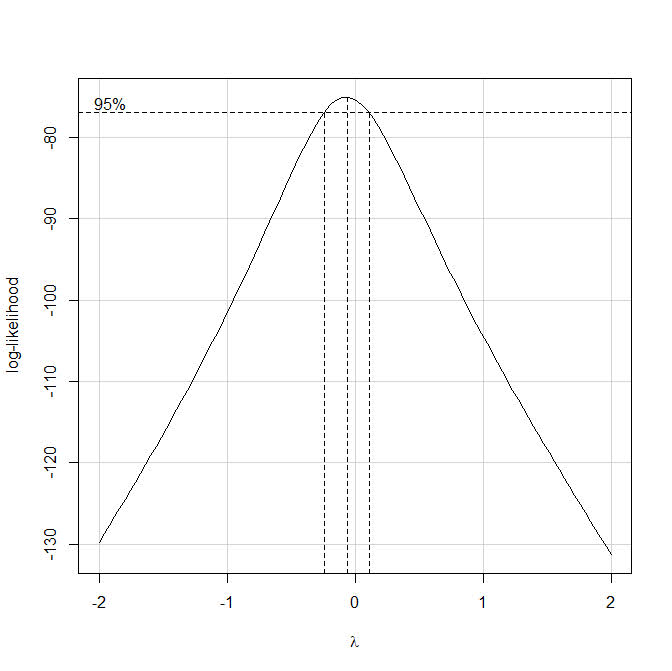So if we look at the `orig_output` object, it gives us the x and y values for the above plot, but it does not give us the dashed line locations in the plot.Typically here I would type out `boxCox` without the parenthesis at the prompt to get the function definition. That does not quite work here, as it is unhelpful and just gets us the message `useMethod(boxCox)`. From here we can do the function `method(boxCox)` to help slightly more – we can see that the `boxCox` function really has 3 different functions, that depend on the original input.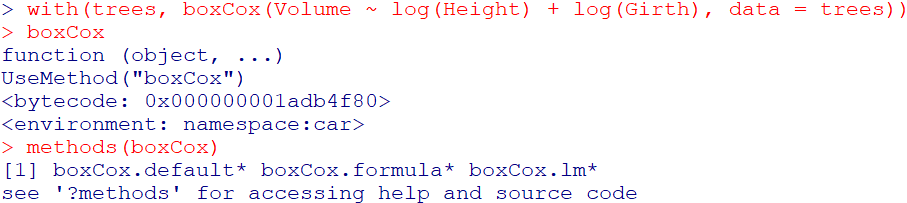Here we are specifying the formula interface to the function call, so lets look at `getAnywhere(boxCox.formula)`: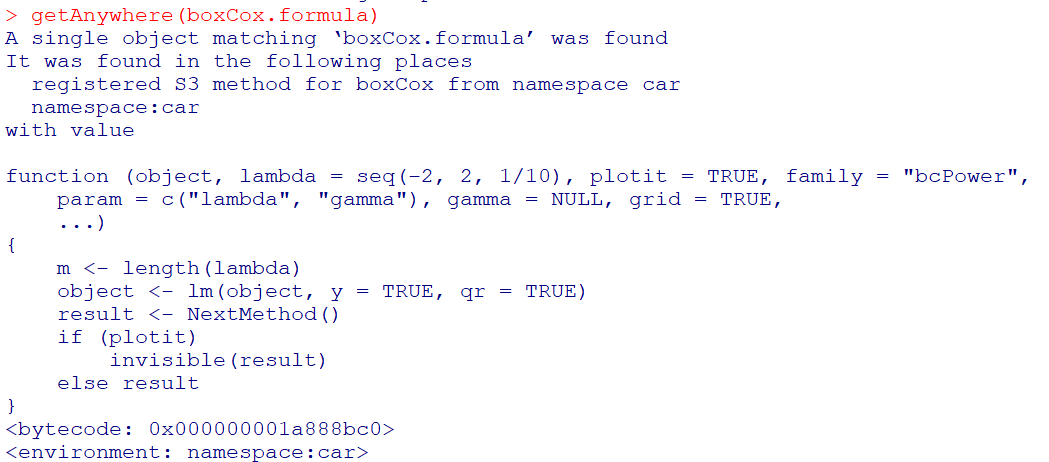Well, that is not very helpful, lets look at `getAnywhere(boxCox.default)` instead: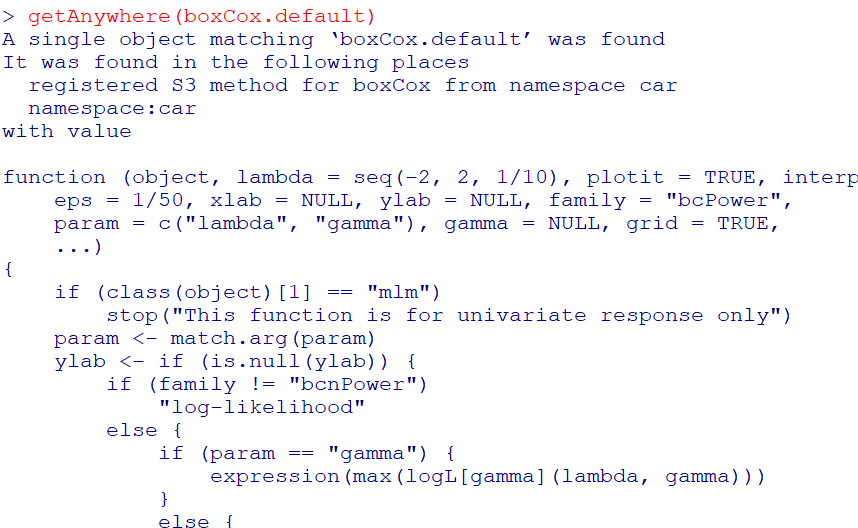Ok, that is what we are going for. If you look into the function, at the very end you will see how it draws those dashed reference lines (anything drawn with `lty = 2` in the code).

So what is happening here is that the different `boxCox` function calls are all daisy chained together, and it goes from formula -> lm object -> the original boxCox function. Now that we can see the function, we can make some small changes to have it return the locations of the vertical/horizontal reference lines that we want (or we could change it to accept a `ylim` argument directly). I name this new function `boxCox.new`.

``````# Modifying the function to return all the info you need
boxCox.new <- function(object, lambda = seq(-2, 2, 1/10), plotit = TRUE, interp = plotit,
eps = 1/50, xlab = NULL, ylab = NULL, family = "bcPower",
param = c("lambda", "gamma"), gamma = NULL, grid = TRUE,
...)
{
if (class(object) == "mlm")
stop("This function is for univariate response only")
param <- match.arg(param)
ylab <- if (is.null(ylab)) {
if (family != "bcnPower")
"log-likelihood"
else {
if (param == "gamma") {
expression(max(logL[gamma](lambda, gamma)))
}
else {
expression(max[lambda](logL(lambda, gamma)))
}
}
}
else ylab
xlab <- if (is.null(xlab)) {
if (param == "lambda")
expression(lambda)
else expression(gamma)
}
else xlab
#fam <- matchFun(family) #Needed to change this to base function
fam <- match.fun(family)
if (is.null(object\$y) || is.null(object\$qr))
stop(paste(deparse(substitute(object)), "does not have both 'qr' and 'y' components"))
y <- object\$y
n <- length(y)
xqr <- object\$qr
xl <- loglik <- if (family != "bcnPower")
as.vector(lambda)
else {
if (param == "lambda")
as.vector(lambda)
else {
if (!is.null(gamma))
as.vector(gamma)
else {
p1 <- powerTransform(object, family = "bcnPower")
gam <- p1\$gamma
se <- sd(y)
seq(max(0.01, gam - 3 * se), gam + 3 * se, length = 100)
}
}
}
m <- length(xl)
if (family != "bcnPower") {
for (i in 1L:m) {
yt <- fam(y, xl[i], j = TRUE)
loglik[i] <- -n/2 * log(sum(qr.resid(xqr, yt)^2))
}
}
else {
lambda.1d <- function(gamma) {
fn <- function(lam) bcnPowerllik(NULL, y, NULL, lambda = lam,
gamma = gamma, xqr = xqr)\$llik
f <- optimize(f = fn, interval = c(-3, 3), maximum = TRUE)
f\$objective
}
gamma.1d <- function(lambda) {
fn <- function(gam) bcnPowerllik(NULL, y, NULL, lambda = lambda,
gamma = gam, xqr = xqr)\$llik
f <- optimize(f = fn, interval = c(0.01, max(y)),
maximum = TRUE)
f\$objective
}
for (i in 1L:m) {
loglik[i] <- if (param == "lambda")
gamma.1d(loglik[i])
else lambda.1d(loglik[i])
}
}
if (interp) {
sp <- spline(xl, loglik, n = 100)
xl <- sp\$x
loglik <- sp\$y
m <- length(xl)
}
if (plotit) {
mx <- (1L:m)[loglik == max(loglik)][1L]
Lmax <- loglik[mx]
lim <- Lmax - qchisq(19/20, 1)/2
# Adding in vector to contain x functions location and top line
xF <- c()
xT <- c()
plot(xl, loglik, xlab = xlab, ylab = ylab, type = "n",
ylim = range(loglik, lim))
if (grid) {
grid(lty = 1, equilogs = FALSE)
box()
}
lines(xl, loglik)
plims <- par("usr")
abline(h = lim, lty = 2)
y0 <- plims[3L]
scal <- (1/10 * (plims[4L] - y0))/par("pin")[2L]
scx <- (1/10 * (plims[2L] - plims[1L]))/par("pin")[1L]
text(xl[1L] + scx, lim + scal, " 95%")
la <- xl[mx]
if (mx > 1 && mx < m)
segments(la, y0, la, Lmax, lty = 2)
xF <- c(xF, la)
xT <- c(xT, Lmax)
ind <- range((1L:m)[loglik > lim])
if (loglik[1L] < lim) {
i <- ind[1L]
x <- xl[i - 1] + ((lim - loglik[i - 1]) * (xl[i] -
xl[i - 1]))/(loglik[i] - loglik[i - 1])
segments(x, y0, x, lim, lty = 2)
xF <- c(xF, x)
xT <- c(xT, lim)
}
if (loglik[m] < lim) {
i <- ind[2L] + 1
x <- xl[i - 1] + ((lim - loglik[i - 1]) * (xl[i] -
xl[i - 1]))/(loglik[i] - loglik[i - 1])
segments(x, y0, x, lim, lty = 2)
xF <- c(xF, x)
xT <- c(xT, lim)
}
# See definitions of hline, vlines, vtop, ybase, just returning that info
return(list(x = xl, y = loglik, hline = lim, vlines = xF, vtop = xT, ybase = y0))
}
list(x = xl, y = loglik)
}``````

But this won’t work offhand with just calling `boxCox.new` with our same prior function calls, so we need to just entirely replace the original `boxCox.default` function for our daisy chain of function references to work. Here can use the `assignInNamespace` function to effectively overwrite the original.

``````# Need to do this to get it to work with lm objects
assignInNamespace("boxCox.default",boxCox.new,ns="car")

r1 <- with(trees, boxCox(Volume ~ log(Height) + log(Girth), data = trees))
r2 <- with(trees, boxCox(V2 ~ log(Height) + log(Girth), data = trees))``````

And now if we inspect either `r1` or `r2` you can see it returns the info we want.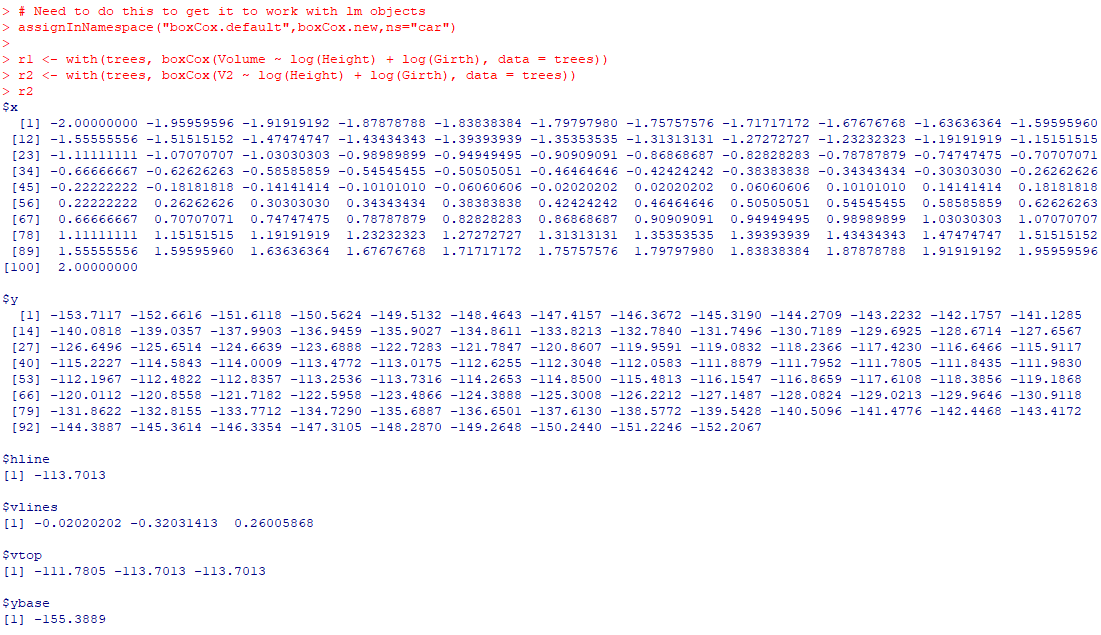And now we build own our set of plots. I don’t have the nice text annotations (or the default grid lines), but leave that to the reader to do that extra work.

``````par(mfrow=c(2,1), mai = c(1, 1, 0.2, 1))
plot(r1\$x,r1\$y,ylim=c(-160,-70), type='l', xaxp = c(-160,-70, 8),
xlab=expression(lambda),ylab='log-Likelihood')
# You need to specify the bottom of the segment to match your limit
abline(h = r1\$hline, lty = 2)
segments(r1\$vlines, -160, r1\$vlines, r1\$vtop, lty = 2)
plot(r2\$x, r2\$y,ylim=c(-160,-70), type='l', xaxp = c(-160,-70, 8),
xlab=expression(lambda),ylab='log-Likelihood')
segments(r2\$vlines, -160, r2\$vlines, r2\$vtop, lty = 2)
abline(h = r2\$hline, lty = 2)``````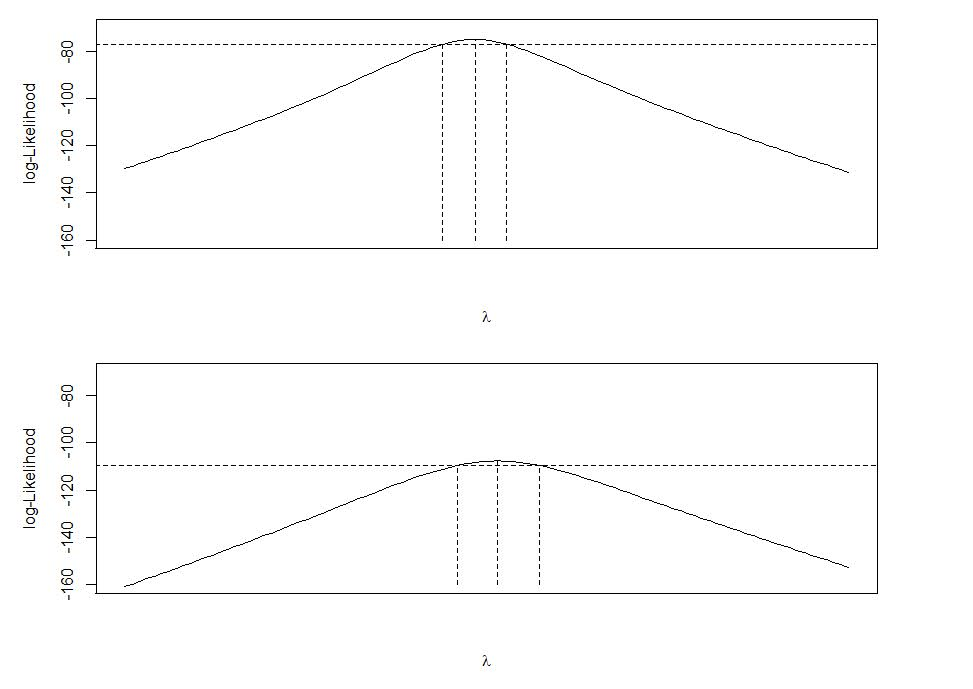I have done this previously for default plots in base R that I wanted to make myself in `ggplot`, which you could do here as well and do a facetted plot instead of the `par` deal with multiple rows (ggplot takes care of the spacing a bit nicer). But that is too much work for this quick tip to cajole those different data frames to do the facets for ggplot.

# Simulating Group Based Trajectories (in R)

The other day I pointed out on Erwin Kalvelagen’s blog how mixture models are a solution to fit regression models with multiple lines (where identification of which particular function/line is not known in advance).

I am a big fan of simulating data when testing out different algorithms for simply the reason it is often difficult to know how an estimator will behave with your particular data. So we have a bunch of circumstances with mixture models (in particular here I am focusing on repeated measures group based traj type mixture models) that it is hard to know upfront how they will do. Do you want to estimate group based trajectories, but have big N and small T? Or the other way, small N and big T? (Larger sample sizes tend to result in identifying more mixtures as you might imagine (Erosheva et al., 2014).) Do you have sparse Poisson data? Or high count Poisson data? Do you have 100,000 data points, and want to know how big of data and how long it may take? These are all good things to do a simulation to see how it behaves when you know the correct answer.

These are relevant no matter what the particular algorithm – so the points are all the same for k-medoids for example (Adepeju et al., 2021; Curman et al., 2015). Or whatever clustering algorithm you want to use in this circumstance. So here I show a few different simulations showing:

• GBTM can recover the correct underlying equations
• AIC/BIC fit stats have a difficult time distinguishing the correct number of groups
• If the underlying model is a random effects instead of latent clusters, AIC/BIC performs quite well

The last part is because GBTM models have a habit of spitting out solutions, even if the true underlying data process has no discrete groups. This is what Skardhamar (2010) did in his article. It was focused on life course, but it applies equally to the spatial analysis GBTM myself and others have done as well (Curman et al., 2015; Weisburd et al., 2004; Wheeler et al., 2016). I’ve pointed out in the past that even if the fit for GBTM looks good, the underlying data can suggest a random effects model will work quite well, and Greenberg (2016) makes pretty much the same point as well.

# Example in R

In the past I have shown how to use the crimCV package to fit these group based traj models, specifically zero-inflated Poisson models (Nielsen et al., 2014). Here I will show a different package, the R `flexmix` package (Grün & Leisch, 2007). This will be Poisson mixtures, but they have an example of doing zip models in there docs if you want.

So first, I load in the `flexmix` library, set the seed, and generate longitudinal data for three different Poisson models. One thing to note here, mixture models don’t assign an observation 100% to an underlying mixture, but the data I simulate here is 100% in a particular group.

``````################################################
library("flexmix")
set.seed(10)

# Generate simulated data
n <- 200 #number of individuals
t <- 10   #number of time periods
dat <- expand.grid(t=1:t,id=1:n)

# Setting up underlying 3 models
time <- dat\$t
p1 <- 3.5 - time
p2 <- 1.3 + -1*time + 0.1*time^2
p3 <- 0.15*time
p_mods <- data.frame(p1,p2,p3)

# Selecting one of these by random
# But have different underlying probs
latent <- sample(1:3, n, replace=TRUE, prob=c(0.35,0.5,0.15))
dat\$lat <- expand.grid(t=1:t,lat=latent)\$lat
dat\$sel_mu <- p_mods[cbind(1:(n*t), dat\$lat)]
dat\$obs_pois <- rpois(n=n*t,lambda=exp(dat\$sel_mu))
################################################``````

Now that is the hard part really – figuring out exactly how you want to simulate your data. Here it would be relatively simple to increase the number of people/areas or time period. It would be more difficult to figure out underlying polynomial functions of time.

Next part we fit a 3 mixture model, then assign the highest posterior probabilities back into the original dataset, and then see how we do.

``````################################################
# Now fitting flexmix model
mod3 <- flexmix(obs_pois ~ time + I(time^2) | id,
model = FLXMRglm(family = "poisson"),
data = dat, k = 3)
dat\$mix3 <- clusters(mod3)

# Seeing if they overlap with true labels
table(dat\$lat, dat\$mix3)/t
################################################``````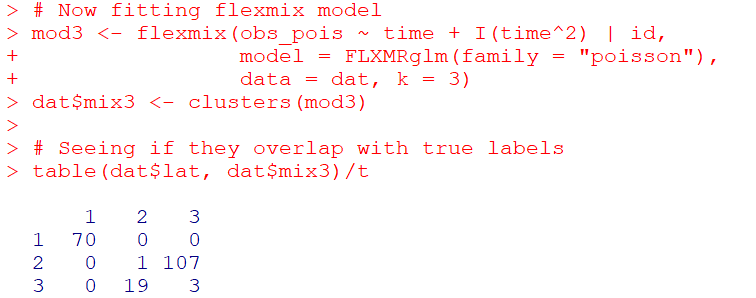So you can see that the identified groupings are quite good. Only 4 groups out of 200 are mis-placed in this example.

Next we can see if the underlying equations were properly recovered (you can have good separation between groups, but the polynomial fit may be garbage).

``````# Seeing if the estimated functions are close
rm3 <- refit(mod3)
summary(rm3)``````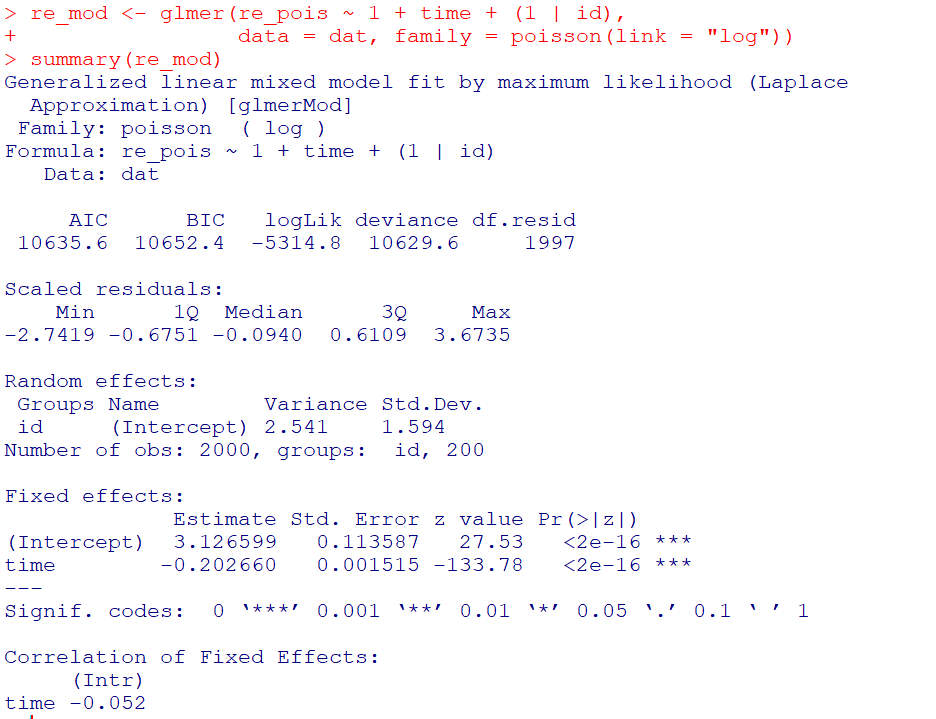This shows the equations are really as good as you could expect. The standard errors are as wide as they are because this isn’t really all that large a data sample for generalized linear models.

So this shows that if I feed in the correct underlying equation (almost, I could technically submit different equations with/without quadratic terms for example). But what about the real world situation in which you do not know the correct number of groups? Here I fit models for 1 to 8 groups, and then use the typical AIC/BIC to see which group it selects:

``````################################################
# If I look at different groups will AIC/BIC
# pick the right one?

group <- 1:8
left_over <- group[!(group %in% 3)]
aic <- rep(-1, 8)
bic <- rep(-1, 8)
aic <- AIC(mod3)
bic <- BIC(mod3)

for (i in left_over){
mod <- flexmix(obs_pois ~ time + I(time^2) | id,
model = FLXMRglm(family = "poisson"),
data = dat, k = i)
aic[i] <- AIC(mod)
bic[i] <- BIC(mod)
}

fit_stats <- data.frame(group,aic,bic)
fit_stats
################################################``````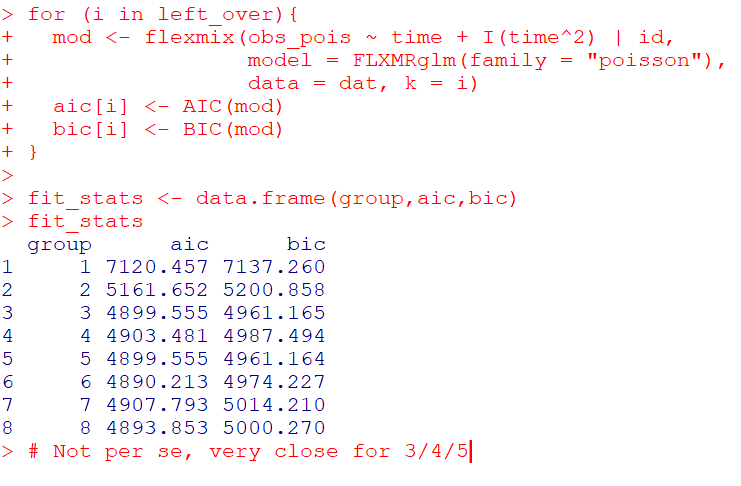Here it actually fit the same model for 3/5 groups (sometimes even if you tell flexmix to fit 5 groups, it will only return a smaller number). You can see that the fit stats for group 4 through are almost the same. So while AIC/BIC did technically pick the right number in this simulated example, it is cutting the margin pretty close to picking 4 groups in this data instead of 3.

So the simulation Skardhamar (2010) did was slightly different than this so far. What he did was simulate data with no underlying trajectory groups, and then showed GBTM tended to spit out solutions. Here I will show that is the case as well. I simulate random intercepts and a simple linear trend over time.

``````################################################
# Simulate random effects model
library(lme4)
rand_eff <- rnorm(n=n,0,1.5)
dat\$re <- expand.grid(t=1:t,re=rand_eff)\$re
dat\$re_pois <- rpois(n=n*t,lambda=exp(dat\$sel_mu))
dat\$mu_re <- 3 + -0.2*time + dat\$re
dat\$re_pois <- rpois(n=n*t,lambda=exp(dat\$mu_re))

re_mod <- glmer(re_pois ~ 1 + time + (1 | id),
data = dat, family = poisson(link = "log"))
summary(re_mod)
################################################``````So you can see that the random effects model is all fine and dandy – recovers both the fixed coefficients, as well as estimates the correct variance for the random intercepts.

So here I go and see how the AIC/BIC compares for the random effects models vs GBTM models for 1 to 8 groups (I stuff the random effects model in the first row for group 0):

``````################################################
# Test AIC/BIC for random effects vs GBTM
group <- 0:8
left_over <- 1:8
aic <- rep(-1, 9)
bic <- rep(-1, 9)
aic <- AIC(re_mod)
bic <- BIC(re_mod)

for (i in left_over){
mod <- flexmix(re_pois ~ time + I(time^2) | id,
model = FLXMRglm(family = "poisson"),
data = dat, k = i)
aic[i+1] <- AIC(mod)
bic[i+1] <- BIC(mod)
}

fit_stats <- data.frame(group,aic,bic)
fit_stats
################################################``````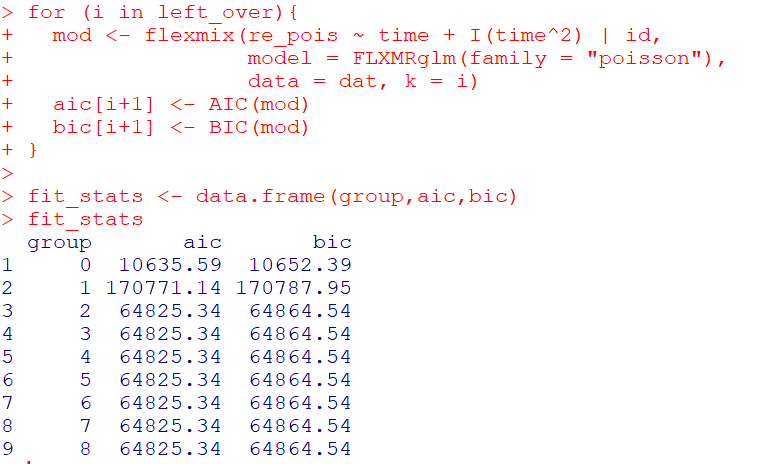So it ends up flexmix will not give us any more solutions than 2 groups. But that the random effect fit is so much smaller (either by AIC/BIC) than the GBTM you wouldn’t likely make that mistake here.

I am not 100% sure how well we can rely on AIC/BIC for these different models (R does not count the individual intercepts as a degree of freedom here, so k=3 instead of k=203). But no reasonable accounting of k would flip the AIC/BIC results for these particular simulations.

One of the things I will need to experiment with more, I really like the idea of using out of sample data to validate these models instead of AIC/BIC – no different than how Nielsen et al. (2014) use leave one out CV. I am not 100% sure if that is possible in this set up with `flexmix`, will need to investigate more. (You can have different types of cross validation in that context, leave entire groups out, or forecast missing data within an observed group.)

# References

Adepeju, M., Langton, S., & Bannister, J. (2021). Anchored k-medoids: a novel adaptation of k-medoids further refined to measure long-term instability in the exposure to crime. Journal of Computational Social Science, 1-26.

Grün, B., & Leisch, F. (2007). Fitting finite mixtures of generalized linear regressions in R. Computational Statistics & Data Analysis, 51(11), 5247-5252.

Curman, A. S., Andresen, M. A., & Brantingham, P. J. (2015). Crime and place: A longitudinal examination of street segment patterns in Vancouver, BC. Journal of Quantitative Criminology, 31(1), 127-147.

Erosheva, E. A., Matsueda, R. L., & Telesca, D. (2014). Breaking bad: Two decades of life-course data analysis in criminology, developmental psychology, and beyond. Annual Review of Statistics and Its Application, 1, 301-332.

Greenberg, D. F. (2016). Criminal careers: Discrete or continuous?. Journal of Developmental and Life-Course Criminology, 2(1), 5-44.

Nielsen, J. D., Rosenthal, J. S., Sun, Y., Day, D. M., Bevc, I., & Duchesne, T. (2014). Group-based criminal trajectory analysis using cross-validation criteria. Communications in Statistics-Theory and Methods, 43(20), 4337-4356.

Skardhamar, T. (2010). Distinguishing facts and artifacts in group-based modeling. Criminology, 48(1), 295-320.

Weisburd, D., Bushway, S., Lum, C., & Yang, S. M. (2004). Trajectories of crime at places: A longitudinal study of street segments in the city of Seattle. Criminology, 42(2), 283-322.

Wheeler, A. P., Worden, R. E., & McLean, S. J. (2016). Replicating group-based trajectory models of crime at micro-places in Albany, NY. Journal of Quantitative Criminology, 32(4), 589-612.

# The spatial dispersion of NYC shootings in 2020

If you had asked me at the start of widespread Covid lockdown measures what the effect would be on crime, I am pretty sure I would have guessed it will make crime go down. Fewer people out and about causes fewer interactions that can lead to a crime. That isn’t how it has shaped up though, quite a few places have seen increases in serious violent crime. One of the most dramatic examples of this is that shootings in NYC doubled from 900 in 2019 to over 1800 in 2020. I am going to show how to generate this chart later via some R code, but it is easier to show than to say. NYPD’s open data on shootings (historical, current) go back to 2006.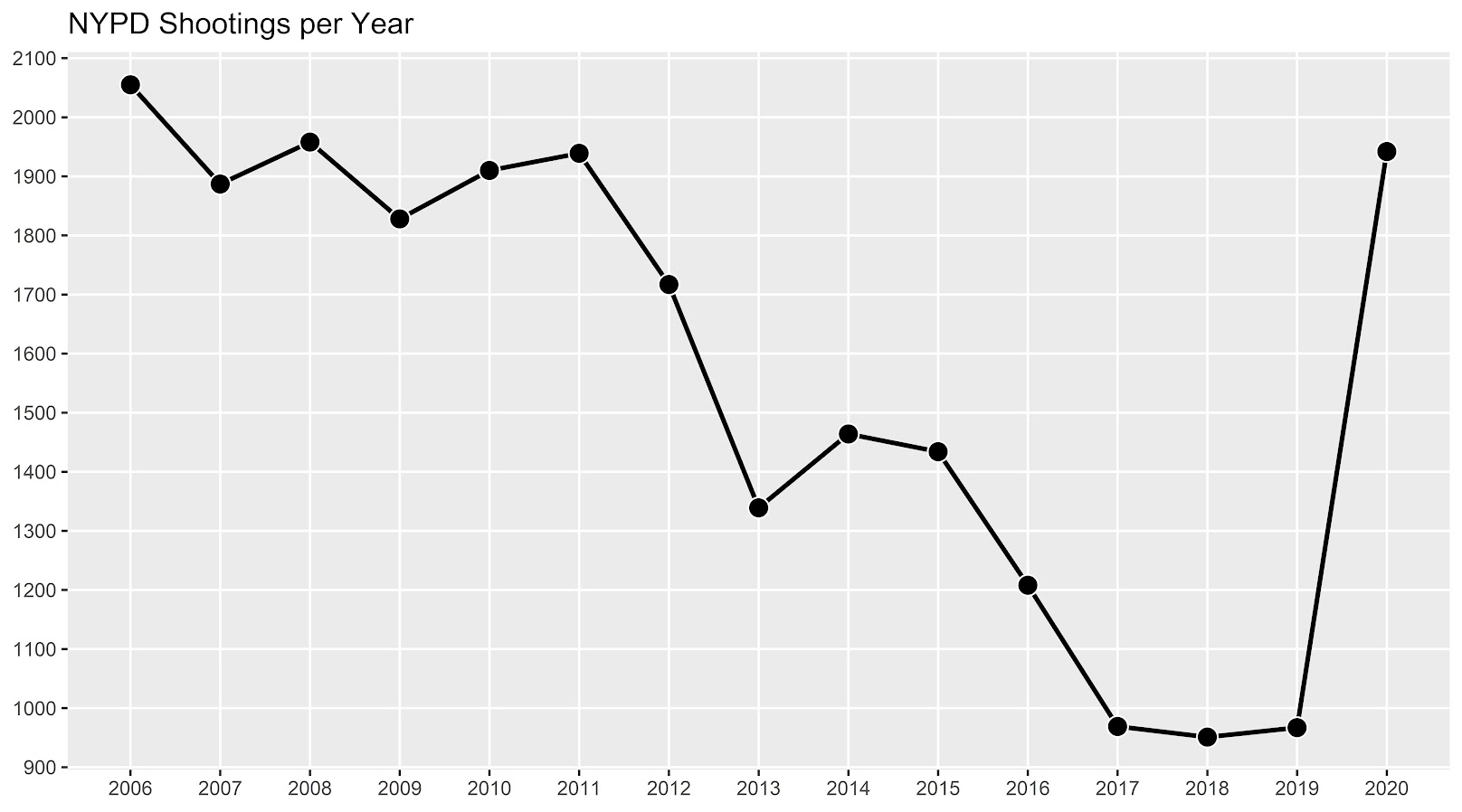I know I am critical on this site of folks overinterpreting crime increases, for example going from 20 to 35 is pretty weak evidence of an increase given the inherent variance for low count Poisson data (a Poisson e-test has a p-value of 0.04 in that case). But going from 900 to 1800 is a much clearer signal.

Jerry Ratcliffe recently posted an R library to do his crime dispersion analysis, so I figured this would be an excellent example use case. The idea behind this analysis is spatial – we know there is a crime increase, but did the increase happen everywhere, or did it just happen in a few locations. Here I am going to use the NYPD shooting data aggregated at the precinct level to test this.

As another note, while I often use micro-spatial units of analysis in my work, this method, along with others (such as the sppt test), are just not going to work out for very low count, very tiny spatial units of analysis. I would suggest offhand to only do this analysis if the spatial units of analysis under study have an average of at least 10 crimes per area in the pre time period. Which is right about on the mark for the precinct analysis in NYC.

Here is the data and R code to follow along, below I will give a walkthrough.

# Crime increase dispersion analysis in R

So first as some front matter, I load in my libraries (Jerry’s `crimedispersion` you can install from github via devtools, see his page for an example), and the function I define here I’ve gone over in a prior blog post of mine as well.

``````###############################
library(ggplot2)
library(crimedispersion)

make_cont <- function(pre_crime,post_crime,levels=c(-3,0,3),lr=10,hr=max(pre_crime)*1.05,steps=1000){
#calculating the overall crime increase
ov_inc <- sum(post_crime)/sum(pre_crime)
#Making the sequence on the square root scale
gr <- seq(sqrt(lr),sqrt(hr),length.out=steps)^2
cont_data <- expand.grid(gr,levels)
names(cont_data) <- c('x','levels')
cont_data\$inc <- cont_data\$x*ov_inc
cont_data\$lines <- cont_data\$inc + cont_data\$levels*sqrt(cont_data\$inc)
return(as.data.frame(cont_data))
}

my_dir <- 'D:\\Dropbox\\Dropbox\\Documents\\BLOG\\NYPD_ShootingIncrease\\Analysis'
setwd(my_dir)
###############################``````

Now we are ready to import our data and stack them into a new data frame. (These are individual incident level shootings, not aggregated. If I ever get around to it I will do an analysis of fatality and distance to emergency rooms like I did with the Philly data.)

``````###############################
# Get the NYPD data and stack it
# From https://data.cityofnewyork.us/Public-Safety/NYPD-Shooting-Incident-Data-Year-To-Date-/5ucz-vwe8
# And https://data.cityofnewyork.us/Public-Safety/NYPD-Shooting-Incident-Data-Historic-/833y-fsy8
# On 2/1/2021

# Just one column off
print( cbind(names(old), names(new)) )
names(new) <- names(old)
shooting <- rbind(old,new)
###############################``````

Now we just want to do aggregate counts of these shootings per year and per precinct. So first I substring out the year, then use `table` to get aggregate counts in R, then make my nice time series graph using ggplot.

``````###############################
# Create the current year and aggregate
shooting\$Year <- substr(shooting\$OCCUR_DATE, 7, 10)
year_stats <- as.data.frame(table(shooting\$Year))
year_stats\$Year <- as.numeric(as.character(year_stats\$Var1))
year_plot <- ggplot(data=year_stats, aes(x=Year,y=Freq)) +
geom_line(size=1) + geom_point(shape=21, colour='white', fill='black', size=4) +
scale_y_continuous(breaks=seq(900,2100,by=100)) +
scale_x_continuous(breaks=2006:2020) +
theme(axis.title.x=element_blank(), axis.title.y=element_blank(),
panel.grid.minor = element_blank()) +
ggtitle("NYPD Shootings per Year")

year_plot
# Not quite the same as Petes, https://copinthehood.com/shooting-in-nyc-2020/
###############################``````Part of the reason I do this is not because I don’t trust Pete’s analysis, but because I don’t want to embed pictures from someone elses website! So wanted to recreate the time series graph myself. So next up we need to do the same aggregating, but not for the whole city, but by each precinct. You can use the same table method again, but simply pass in additional columns. That gets you the data in long format, so then I reshape it to wide for later analysis (so each row is a single precinct and each column is a yearly count of shootings). (Note there have been some splits in precincts over the years IIRC, I don’t worry about that here, will cause it to be 0,0 in the 2019/2020 data I look at.)

``````###############################
#Now aggregating to year and precinct
counts <- as.data.frame(table(shooting\$Year, shooting\$PRECINCT))
names(counts) <- c('Year','PCT','Count')
# Reshape long to wide
count_wide <-  reshape(counts, idvar = "PCT", timevar = "Year", direction = "wide")
###############################``````

And now we can give Jerry’s package a test run, where you just pass it your variable names.

``````# Jerrys function for crime increase dispersion
output <- crimedispersion(count_wide, 'PCT', 'Count.2019', 'Count.2020')
output``````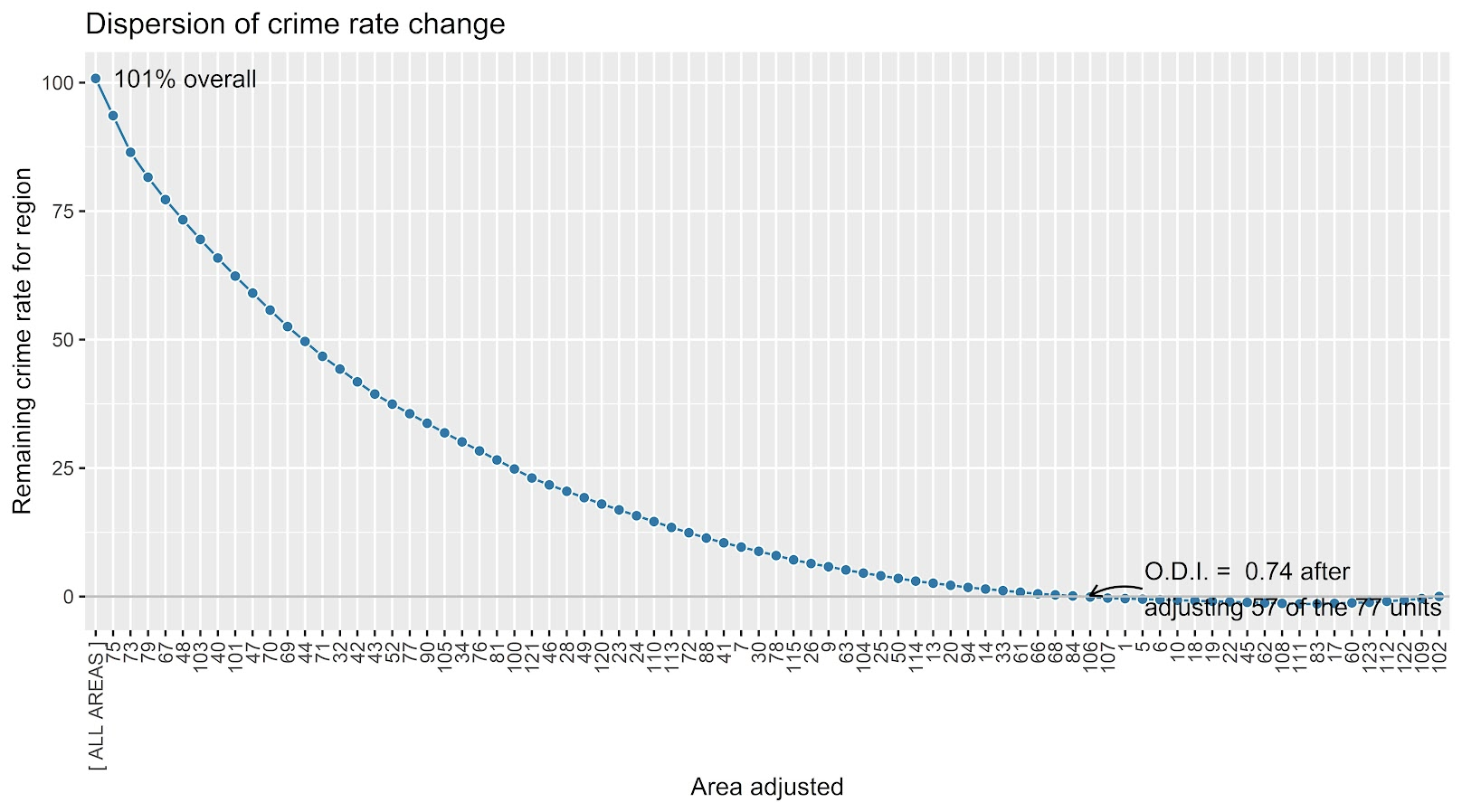The way to understand this is in a hypothetical world in which we could reduce shootings in one precinct at a time, we would need to reduce shootings in 57 of the 77 precincts to reduce 2020 shootings to 2019 levels. So this suggests very widespread increases, it isn’t just concentrated among a few precincts.

Another graph I have suggested to explore this, while taking into account the typical variance with Poisson count data, is to plot the pre crime counts on the X axis, and the post crime counts on the Y axis.

``````###############################
# My example contour with labels
cont_lev <- make_cont(count_wide\$Count.2019, count_wide\$Count.2020, lr=5)

eq_plot <- ggplot() +
geom_line(data=cont_lev, color="darkgrey", linetype=2,
aes(x=x,y=lines,group=levels)) +
geom_point(data=count_wide, shape = 21, colour = "black", fill = "grey", size=2.5,
alpha=0.8, aes(x=Count.2019,y=Count.2020)) +
scale_y_continuous(breaks=seq(0,140,by=10) +
scale_x_continuous(breaks=seq(0,70,by=5)) +
coord_cartesian(ylim = c(0, 140)) +
xlab("2019 Shootings Per Precinct") + ylab("2020 Shootings")
eq_plot
###############################``````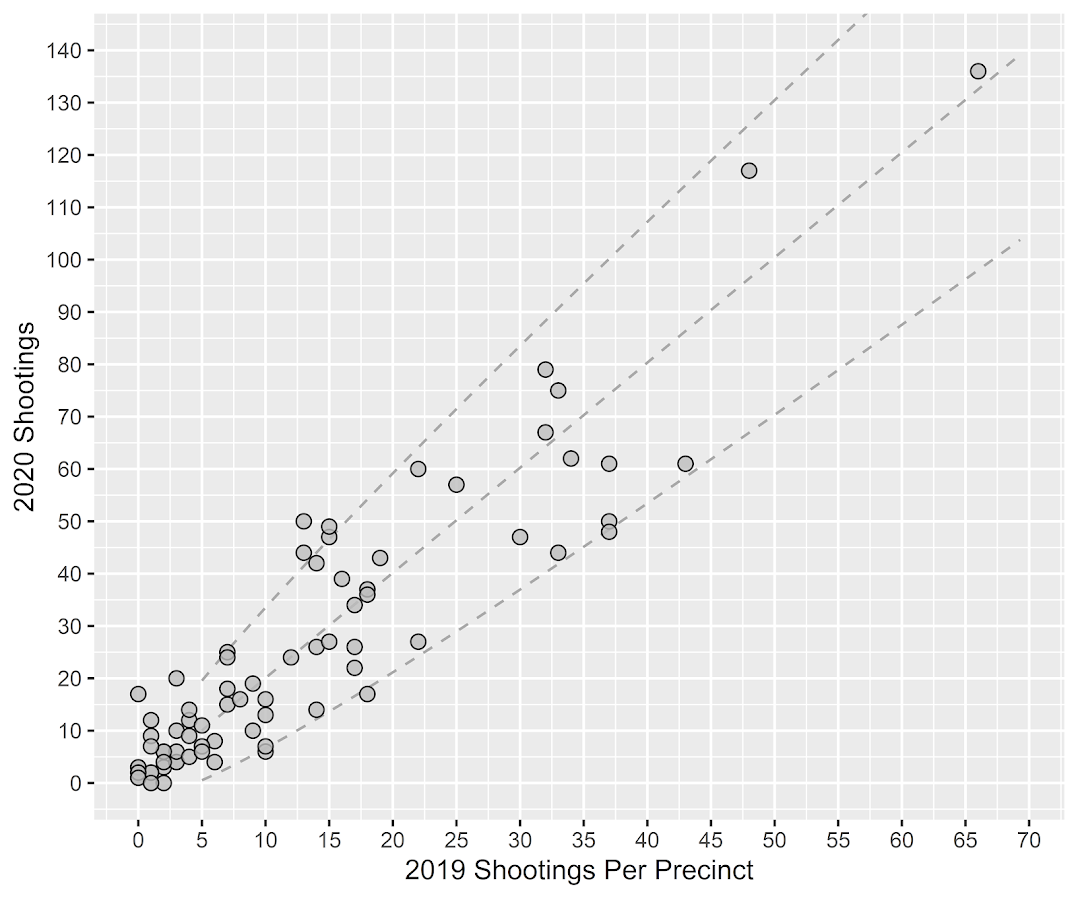The contour lines show the hypothesis that crime increased (by around 100% here). So if a point is near the middle line, it follows that doubled mark almost exactly. The upper/lower lines indicate the typical variance, which is a very good fit to the data here you can see. Very few points are outside the boundaries.

Both of these analyses point to the fact that shooting increases were widespread across NYC precincts. Pretty much everywhere doubled in the number of shootings, it is just some places had a larger baseline to double than others (and the data has some noise, you can pick out some places that did not increase if you cherry pick the data).

And as a final R note, if you want to save these graphs as a nice high resolution PNG, here is an example with Jerry’s dispersion object:

``````# Saving dispersion plot as a high res PNG
png(file = "ODI.png", bg = "transparent", height=5, width=9, units="in", res=1000, type="cairo")
output #this is the object from Jerrys crimedispersion() function earlier
dev.off()``````

Going forward I am wondering if there is a good way to do spatial monitoring for crime data like this, like some sort of control chart that takes into account both space and time. So isn’t retrospective a year later recap, but in near real time identify spatial increases.

# Other References of Interest

• Justin Nix & company have a few blog posts looking at NYC data as well. In the first they talk about the variance in cities, many are up but several are down as well in violence. A later post though updated with the clear increase in shootings in NYC.
• There are too many papers at this point for me to do a bibliography of all the Covid and crime updates, but two open examples are Matt Ashby did a paper on several US cities, and Campedelli et al have an analysis of Chicago. Each show variance again, so no universal up or down in trends, but various examples of increases or decreases both between cities and between different crime types within a city.

# Checking a Poisson distribution fit: An example with officer involved shooting deaths WaPo data (R functions)

So besides code on my GitHub page, I have a list of various statistic functions I’ve scripted on the blog over the years on my code snippets page. One of those functions I will illustrate today is some R code to check the fit of the Poisson distribution. Many of my crime analysis examples rely on crime data being approximately Poisson distributed. Additionally it is relevant in regression model building, e.g. should I use a Poisson GLM or do I need to use some type of zero-inflated model?

Here is a brief example to show how my R code works. You can source it directly from my dropbox page. Then I generated 10k simulated rows of Poisson data with a mean of 0.2. So I see many people in CJ make the mistake that, OK my data has 85% zeroes, I need to use some sort of zero-inflated model. If you are working with very small spatial/temporal units of analysis and/or rare crimes, it may be the mean of the distribution is quite low, and so the Poisson distribution is actually quite close.

``````# My check Poisson function
source('https://dl.dropboxusercontent.com/s/yj7yc07s5fgkirz/CheckPoisson.R?dl=0')

# Example with simulated data
set.seed(10)
lambda <- 0.2
x <- rpois(10000,lambda)
CheckPoisson(x,0,max(x),mean(x))``````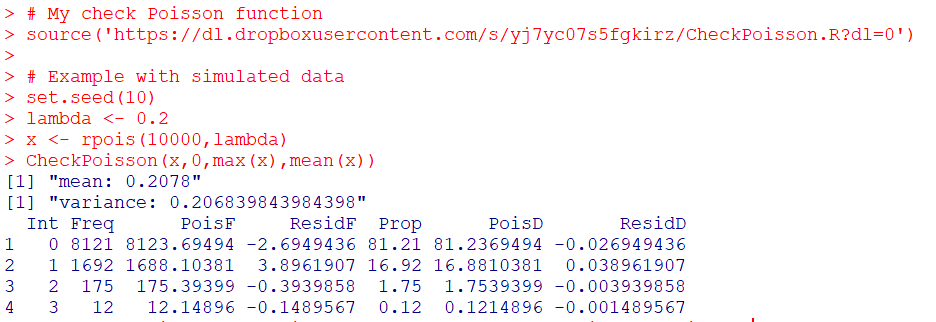Here you can see in the generated table from my `CheckPoisson` function, that with a mean of 0.2, we expect around 81.2% zeroes in the data. And since we simulated the data according to the Poisson distribution, that is what we get. The table shows that out of the 10k simulation rows, 8121 were 0’s, 1692 rows were 1’s etc.

In real life data never exactly conform to hypothetical distributions. But we often want to see how close they are to the hypothetical before building predictive models. A real life example as close to Poisson distributed data as I have ever seen is the Washington Post Fatal Use of Force data. Every year WaPo has been collating the data, the total number of Fatal uses of Police Force in the US have been very close to 1000 events per year. And even in all the turmoil this past year, that is still the case.

``````# Washington Post Officer Involved Shooting Deaths Data
stringsAsFactors = F)

# Year Stats
oid\$year <- as.integer(substr(oid\$date,1,4))
year_stats <- table(oid\$year)[1:6]
year_stats
mean(year_stats)
var(year_stats)``````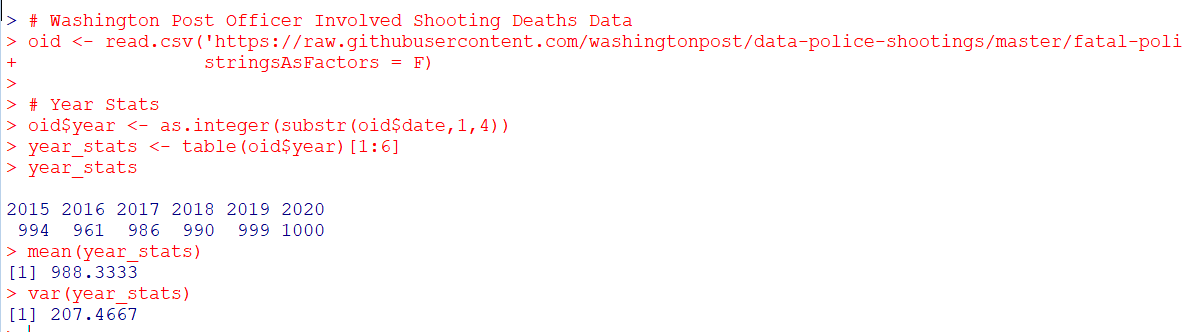One way to check the Poison distribution is that the mean and the variance should be close, and here at the yearly level the data have some evidence of underdispersion according to the Poisson distribution (most crime data is overdispersed – the variance is much greater than the mean). If the actual mean is around 990, you would expect typical variations of say around plus/minus 60 per year (`~ 2*sqrt(990)`). But that only gives us a few observations to check (6 years). We can dis-aggregate the data to smaller intervals and check the Poisson assumption. Here I aggregate to days (note that this includes zero days in the table levels calculation). Then we again check the fit of the Poisson distribution.

``````#Now aggregating to count per day
oid\$date_val <- as.Date(oid\$date)
date_range <- paste0(seq(as.Date('2015-01-01'),max(oid\$date_val),by='days'))
day_counts <- as.data.frame(table(factor(oid\$date,levels=date_range)))
pfit <- CheckPoisson(day_counts\$Freq, 0, 10, mean(day_counts\$Freq))
pfit``````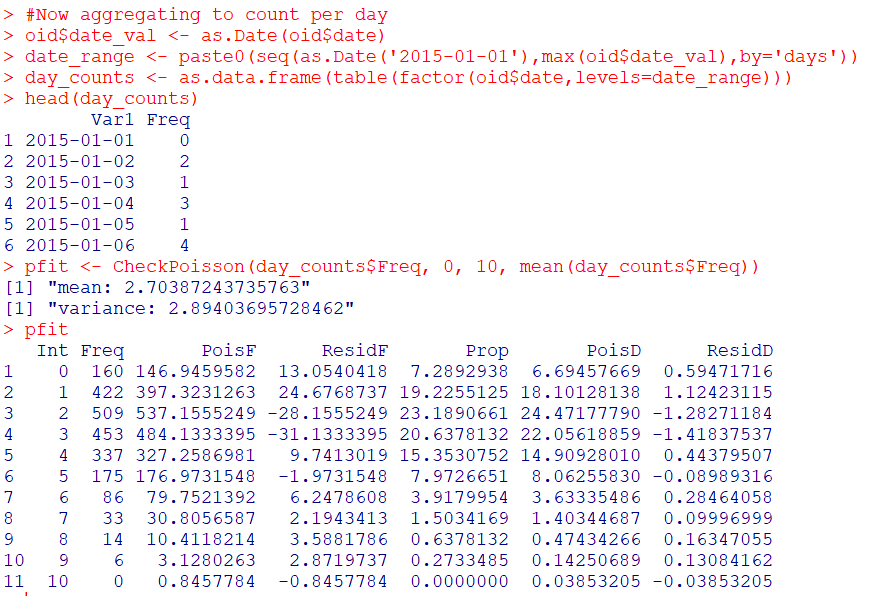According to the mean and the variance, it appears the distribution is a very close fit to the Poisson. We can see in this data we expected to have around 147 days with 0 fatal encounters, and in reality there were 160. I like seeing the overall counts, but another way is via the proportions in the final three columns of the table. You can see for all of the integers, we are less than 2 percentage points off for any particular integer count. E.g. we expect the distribution to have 3 fatal uses of force on about 22% of the days, but in the observed distribution days with 3 events only happened around 21% of the days (or `20.6378132` without rounding). So overall these fatal use of force data of course are not exactly Poisson distributed, but they are quite close.

So the Poisson distribution is motivated via a process in which the inter-arrival dates of events being counted are independent. Or in more simple terms one event does not cause a future event to come faster or slower. So offhand if you had a hypothesis that publicizing officer fatalities made future officers more hesitant to use deadly force, this is not supported in this data. Given that this is officer involved fatal encounters in the entire US, it is consistent with the data generating process that a fatal encounter in one jurisdiction has little to do with fatal encounters in other jurisdictions.

(Crime data we are often interested in the opposite self-exciting hypothesis, that one event causes another to happen in the near future. Self-excitation would cause an increase in the variance, so the opposite process would result in a reduced variance of the counts. E.g. if you have something that occurs at a regular monthly interval, the counts of that event will be underdispersed according to a Poisson process.)

So the above examples just checked a univariate data source for whether the Poisson distribution was a decent fit. Oftentimes academics are interested in whether the conditional distribution is a good fit post some regression model. So even if the marginal distribution is not Poisson, it may be you can still use a Poisson GLM, generate good predictions, and the conditional model is a good fit for the Poisson distribution. (That being said, you model has to do more work the further away it is from the hypothetical distribution, so if the marginal is very clearly off from Poisson a Poisson GLM probably won’t fit very well.)

My `CheckPoisson` function allows you to check the fit of a Poisson GLM by piping in varying predicted values over the sample instead of just one. Here is an example where I use a Poisson GLM to generate estimates conditional on the day of the week (just for illustration, I don’t have any obvious reason fatal encounters would occur more or less often during particular days of the week).

``````#Do example for the day of the week
day_counts\$wd <- weekdays(as.Date(day_counts\$Var1))
mod <- glm(Freq ~ as.factor(wd) - 1, family="poisson", data=day_counts)
#summary(mod), Tue/Wed/Thu a bit higher
lin_pred <- exp(predict(mod))
pfit_wd <- CheckPoisson(day_counts\$Freq, 0, 10, lin_pred)
pfit_wd``````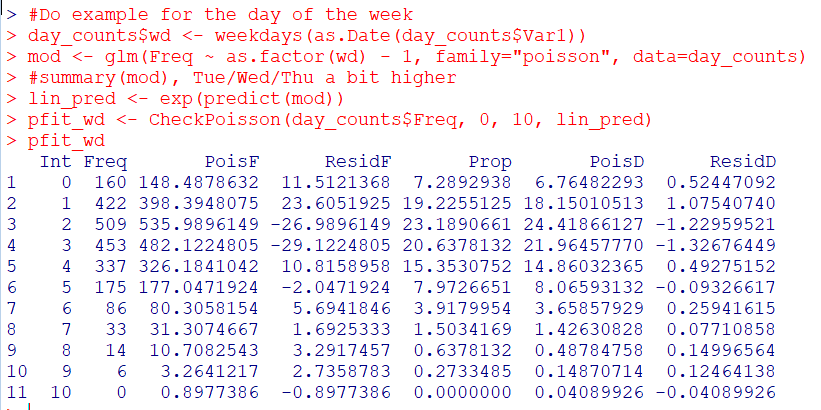You can see that the fit is almost exactly the same as before with the univariate data, so the differences in days of the week does not explain most of the divergence from the hypothetical Poisson distribution, but again this data is already quite close to a Poisson distribution.

So it is common for people to do tests for goodness-of-fit using these tables. I don’t really recommend it – just look at the table and see if it is close. Departures from hypothetical can inform modeling decisions, e.g. if you do have more zeroes than expected than you may need a negative binomial model or a zero-inflated model. If the departures are not dramatic, variance estimates from the Poisson assumption are not likely to be dramatically off-the-mark.

But if you must, here is an example of generating a Chi-Square goodness-of-fit test with the example Poisson fit table.

``````# If you really want to do a test of fit
chi_stat <- sum((pfit\$Freq - pfit\$PoisF)^2/pfit\$PoisF)
df <- length(pfit\$Freq) - 2
dchisq(chi_stat, df)``````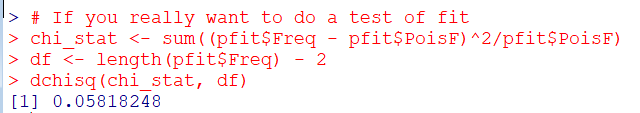So you can see in this example the p-value is just under 0.06.

I really don’t recommend this though for two reasons. One is that with null hypothesis significance testing you are really put in a position that large data samples always reject the null, even if the departures are trivial in terms of the assumptions you are making for whatever subsequent model. The flipside of this is that with small samples the test is underpowered, so there are never many good scenarios where it is useful in practice. Two, you can generate superfluous categories (or collapse particular categories) in the Chi-Square test to increase the degrees of freedom and change the p-value.

One of the things though that this is useful for is checking the opposite, people fudging data. If you have data too close to the hypothetical distribution (so very high p-values here), it can be evidence that someone manipulated the data (because real data is never that close to hypothetical distributions). A famous example of this type of test is whether Mendel manipulated his data.

I intentionally chose the WaPo data as it is one of the few that out of the box really appears to be close to Poisson distributed in the wild. One of my next tasks though is to do some similar code for negative binomial fits. Like Paul Allison, for crime count data I rarely see much need for zero-inflated models. But while I was working on that I noticed that the parameters in NB fits with even samples of 1,000 to 10,000 observations were not very good. So I will need to dig into that more as well.

# Changepoints in CCTV Effects

So I am a big fan of using splines in regression equations to model non-linear effects. But a limitation of these is that you need to upfront say how many knots you want, as well as where the knots are. So I have explored a bit on fitting models that can identify the changepoints themselves. It was a tricky road, I tried building some in deep learning using pytorch, then tried variational auto-encoders in pyro, then pystan (marginalizing the changepoint out), and then pymc3 (using different samplers). All of my attempts failed! But when I used the R mcp library (Lindeløv, 2020), it was able to find my changepoint using simulated data. (It uses JAGS under the hood, no idea why JAGS behaved better than my other attempts.)

# Usecase: Dropoff effect of CCTV on clearance rates

So in spatial criminology, a popular hypothesis is estimating distance decay effects. Ratcliffe (2012) was the first example of using a changepoint regression model to do this, showing a changepoint in the effect of bars on the spatial density of crime nearby. This has been replicated in Xu & Griffiths (2017), and in my work using machine learning and partial dependence plots I show similar changepoint patterns as well (Wheeler & Steenbeek, 2020).

One example use case though I want to mention is not in terms of estimating the spatial density of crime, but with the characteristics of the crime events themselves. Sometimes people I think mistakenly think since I have spatial data, I need to aggregate it to some areal unit, and then do analysis of that areal unit data. That approach is not per-se wrong, but is sometimes a step removed from what you want, and can result in some tricky inferences.

Take for example a recent paper looking at clearances and using RTM by Kennedy et al. (2020). What they do is spatially aggregate homicides cleared and homicides not cleared, and run RTM on each. You might be tempted to interpret if a factor is selected for both models that it does not impact clearances, but it also depends on the size of the effect. So for example, in Brooklyn for drug markets they report a rate ratio of 3.1 and 2.4 (both at the same spatial distance). To translate this into a clearance rate, you need to add the two density estimates for all cases, and then take the cleared cases as the numerator.

``````# Example R code
clear <- exp(-0.1 + log(3.1))
nonclear <- exp(-0.1 + log(2.4))
prop <- clear/(clear + nonclear)
prop #0.5636364``````

Here I am treating `-0.1` as the intercept. So here this is lower, but close to the overall clearance in Brooklyn, 58%. This 56% will be the estimate iff the intercept for each equation is the same, if they are not though it could change the clearance rate estimate either way. Since the Kennedy paper did not report this, we cannot know. So for instance, if we change the intercept estimates so clearances are higher and non-clearances are lower, we get an estimate that drug markets increase clearances slightly, not decrease them:

``````clear <- exp(-0.05 + log(3.1))
nonclear <- exp(-0.2 + log(2.4))
prop <- clear/(clear + nonclear)
prop #0.6001124``````

In this example it probably won’t push them too far either way, but takes a bit of work going from the aggregate data analysis to the estimate we want, how those spatial risk factors impact the clearance rate. There is an easier way though – just incorporate your spatial features, such as the distance the nearest crime generator factor, and estimate a model on the micro level incident data. This is what Kennedy et al. (2020) do later in the paper when incorporating the RTM predictions – I just think they should have done the RTM machinery directly on this problem, instead of the two-step approach.

Examples of my work I have done this approach in the past (incorporating spatial data into the micro level incidents) is with fatalities from gun shot wounds (Circo & Wheeler, 2020). We actually investigated non-linear effects though of distance/drive-time, and did not find evidence of that. Going back to the crime clearance example though, another pre-print I examine the effects of CCTV cameras and find a diminishing effect of case clearances given the distance to the camera (Jung & Wheeler, 2019).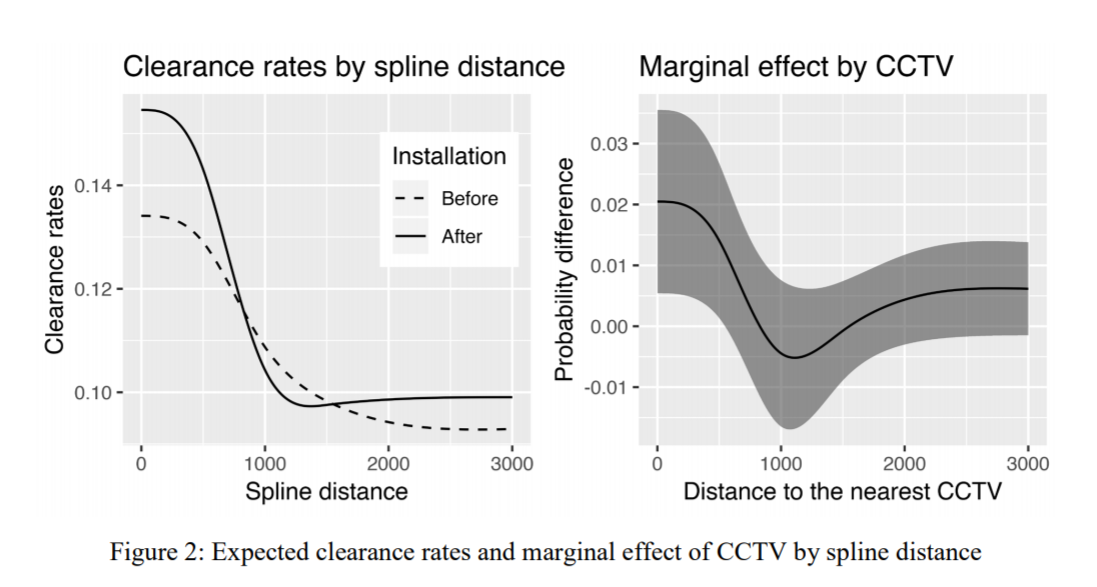So here we use a pre-post design to show there are some selection effects, and we do further analysis to show this camera bump in clearances is only limited to thefts. But we set the splines at 500, 1000, and 1500 feet pre-emptively for the analysis. A reviewer critique of this is that those three locations are arbitrary (which is correct), so here I will see if I do a changepoint model that allows us to find the knot locations if it will show the same ones.

The idea behind this analysis is that CCTV are often used in investigations. Yeondae is an officer in Korea, same as here in the states first things detectives do is to go and grab CCTV footage. Analysis of cameras are often aggregated to their viewsheds, but I think estimating distance decay effects make as much sense. So events closer to the cameras presumably will provide more clear evidence than events at the border of the viewshed. A second point is that even if the event takes place off-camera, there may be evidence cross by the camera viewshed. Detectives will often try to follow individuals across multiple cameras. So both of those factors suggest a distance decay effect both within a cameras viewshed and a decaying effect even outside of the viewshed. (In addition to this, geo coordinates of crime locations are not perfectly accurate measures either, so that could cause effects outside of the viewshed as well.)

Here I am just limiting the data to the post camera data within 3000 feet for thefts, which still is over 26,000 observations. I’ve posted the data/code to follow along here.

# Analysis using mcp in R

Again given my hardship in coding this up myself in python, I created a simulated data example and checked the results using mcp (which you can check in my code). Since mcp recovered my simulated changepoint, (and my python attempts did not), going to go ahead with the mcp library! First, we will import my clearance data and get rid of a few missing cases.

``````#################
library(mcp)
library(ggplot2)
set.seed(10)
#can see I planned on doing this in pytorch at first!
setwd('D:\\Dropbox\\Dropbox\\Documents\\BLOG\\changepoint_pytorch\\Analysis')
theft_clear <- theft_clear[complete.cases(theft_clear),]
#################``````

So first for a reference, if I assume there is a linear changepoint at 1000 feet, here are what my results look like. Note here that this is not aggregated data to spatial locations, each row in this dataset is a theft offense, whether it was cleared, and the distance to the nearest CCTV camera.

``````#################
#What are the coefficients if assume a changepoint of 1000 feet
theft_clear\$x_dif <- (theft_clear\$CAM.DIST - 1000)*(theft_clear\$CAM.DIST > 1000)
theft_mod <- glm(formula = 'STATUSi ~ CAM.DIST + x_dif', family = "binomial", data = theft_clear)
summary(theft_mod) #This gives an estimate of
#################``````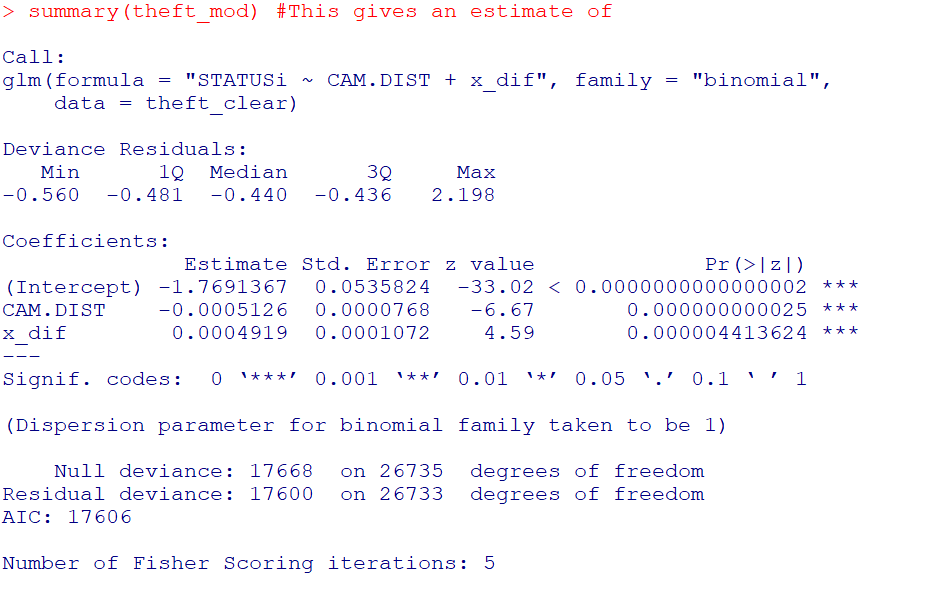And here you can visualize the results alittle easier than trying to back out probabilities for the regression equation:

``````#################
pred_mod <- predict(theft_mod,type='response')
plot(theft_clear\$CAM.DIST,pred_mod, main="Changepoint at 1000 ft",
xlab="Distance from Camera (ft)", ylab="Probability Clearance")
#################``````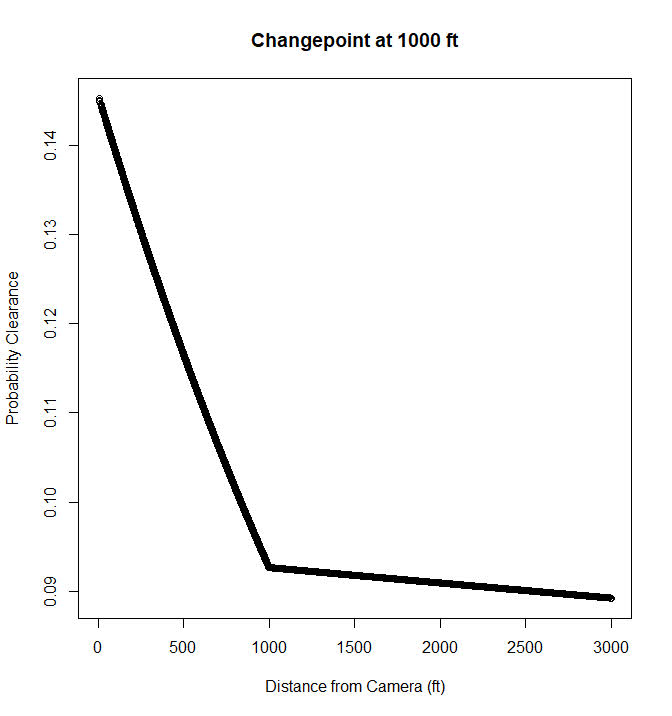So this shows clearances nearby cameras in Dallas are around 15%, and they trail off to around 9% at 1000 feet. After that they continue to tail off, but are nearly flat. But again that is assuming a change point at 1000 feet. But the mcp package lets us actual estimate the changepoint itself using Bayesian regression. Here is the set up that is equivalent to my formulation earlier, in that the changepoint cannot be discontinuous.

``````#################
theft_clear\$x <- theft_clear\$CAM.DIST
model = list(
STATUSi | trials(const) ~ 1 + x,
~ 0 + x  #joined changing rate
)

fit = mcp(model, data = theft_clear, family = binomial(), iter = 3000, adapt = 500)
#################``````

And then if you are following along you can go ahead and take a nap (maybe took 2 hours on my machine?), and when we get back `summary(fit)` gives us: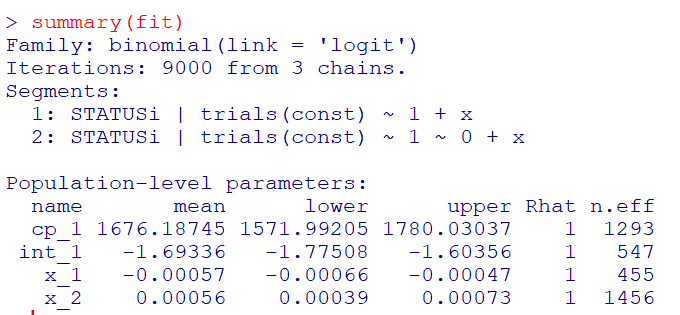So we have very similar coefficients to the manual changepoint model earlier, but the changepoint is around 1600 feet, not 1000. (Although note these are Bayesian credible intervals, not frequentist confidence intervals.) And now to make a nice plot of the fitted model.

``````#Fitted values for new data
newdat <- data.frame(x = (0:300)*10)
newdat\$const <- 1
newdat\$CAM.DIST <- newdat\$x
res <- fitted(fit, newdata = newdat)

p_pred <- ggplot(data=res) +
geom_line(size=1.2, color='black', aes(x = x, y = fitted)) +
geom_ribbon(alpha=0.5, fill='black', aes(x = x, ymin=Q2.5 , ymax=Q97.5)) +
scale_x_continuous(name="Feet from Camera",breaks=seq(0,3000,500),minor_breaks=NULL) +
scale_y_continuous(name="P(Clearance)",breaks=seq(0.06,0.16,0.02),minor_breaks=NULL) +
theme_bw() + theme(panel.grid.major = element_line(colour = 'grey', linetype = 'dashed', size=0.1)) +
theme(text = element_text(size=20))

p_pred``````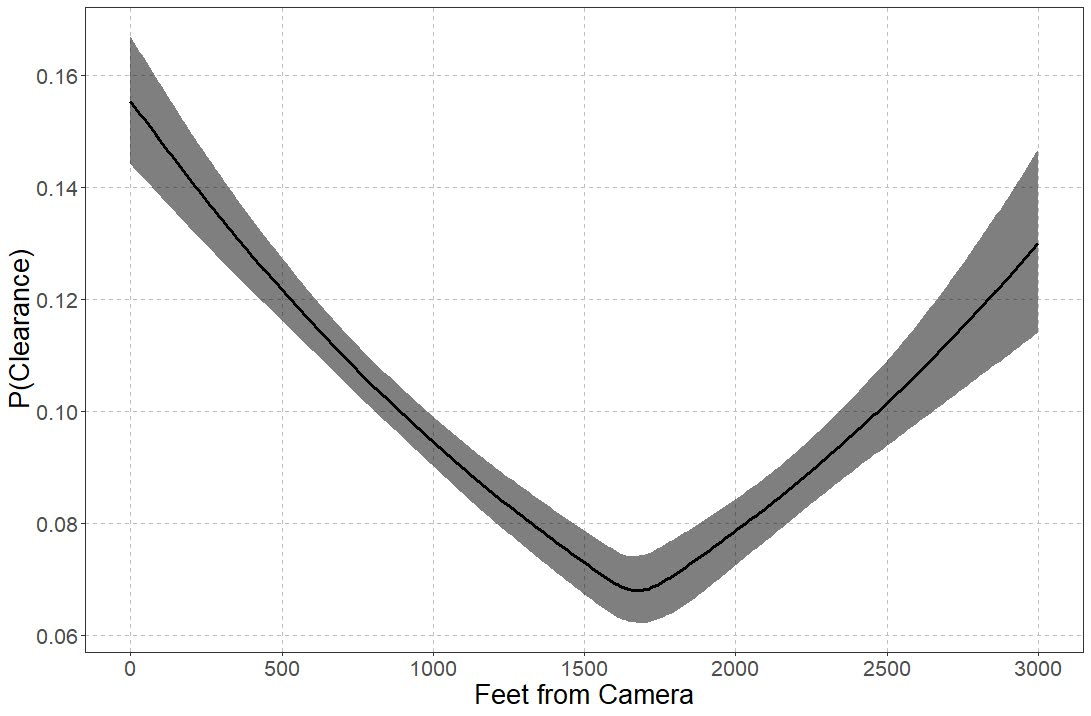So you can see that here it is a nearly linear drop off until 1600 feet, and then starts to climb back up. The climb up I think is likely due to selection effects, but we can’t 100% rule out displacement effects. Displacement effects could occur with cameras if detectives prioritize events around cameras and de-prioritize other events not nearby cameras. Skeptical that applies to thefts in Dallas though, as they very rarely will be assigned a detective at all.

# Wrap Up

So this ended up taking me for a few different turns. One of the things I wanted to be able to test multiple changepoints, maybe if I can ever get pymc3 to give me a reasonable fit, this example is a good illustration. That should also maybe say if you should have no changepoint as well. I think maybe it is much harder to fit those models with binomial data though than with continuous (maybe good for another blog post as well, did simulations at first with 1000 observations and that was a bad idea).

One thing that would be good for evaluating whether change points are reasonable are out of sample predictive comparisons. So say estimate a no changepoint model, a linear changepoint model, and then a model with fixed spline locations. Then see which of those better fits the out of sample data. But since this is a blog post, will leave it as is. But this is a simple illustration to extend prior spatial analysis of changepoints in distance decay effects to one example – crime clearances and CCTV cameras – that I think makes alot of sense.